81% OFF online library of tutorials for mastering Google Sheets

One time purchase \$199

Hey there! Register a new account to access the full suite of features, tutorials, and more..

# VLOOKUP

Use VLOOKUP() when you want a specific value in a column of data. Use VLOOKUP() when you want to retrieve information from a different column based on that value. Great for creating amazing summaries, dynamic reports, or managing inventory

# SUMIF

When you need to calculate the sum of values in a range of cells that meet specific criteria, use SUMIF(). Great to use when you need to filter data. For instance, you can use it to sum all the revenue generated from a particular region, all the orders placed by a specific customer, or all the products with a certain attribute.

# COUNTIF

Use COUNTIF() when you're trying to figure out frequency. Learn how to use COUNTIF in Google Sheets. Also check out it's possible alternative: SUMIF. Depending on what you're trying to do, COUNTIF could be your solution or not.

# IF

Powerful and mysterious. Use IF() to make your spreadsheets gateways. Learn how to use IF in Google Sheets. Also check out it's oft-partner ISBLANK()

# FILTER

the FILTER() formula in Google Sheets allows you to filter data based on a set of criteria. Learn how to use FILTER in Google Sheets. Also check out it's possible combination alternative: INDEX/SORT.

# INDEX

A seemingly innocent and useless formula can be so powerful. INDEX pairs nicely with MATCH to replace VLOOKUP. And it's uses are almost endless. Learn more about the INDEX() formula in Google Sheets.

# VLOOKUP

Powerful vlookup and easy to use. Learn how to use VLOOKUP in Google Sheets. Also check out it's possible alternative: INDEX/MATCH.

# SUMIF

Don't know the syntax, or how to use SUMIF? Learn how to use SUMIF in Google Sheets. Also check out it's possible alternative: COUNTIF. Depending on what you're trying to do, SUMIF could be your solution or not.

# CONCATENATE

Probably the most easily misspelled formula in all of Google Sheets. Learn how to use CONCATENATE in Google Sheets. Also check out it's possible alternative: JOIN.

# 515Formulas

Every Formula in Google Sheets, From ABS to ZTEST, including Vlookup and, my favorite, IF()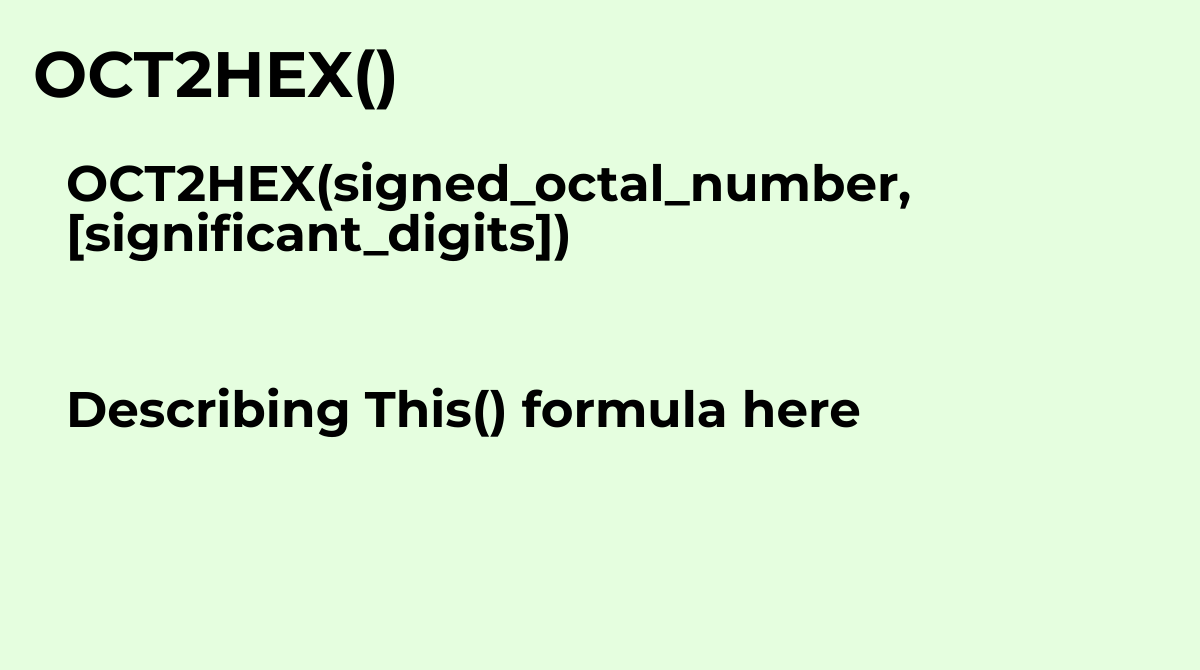Converts a signed octal number to signed hexadecimal format.
`OCT2HEX(signed_octal_number, [significant_digits])`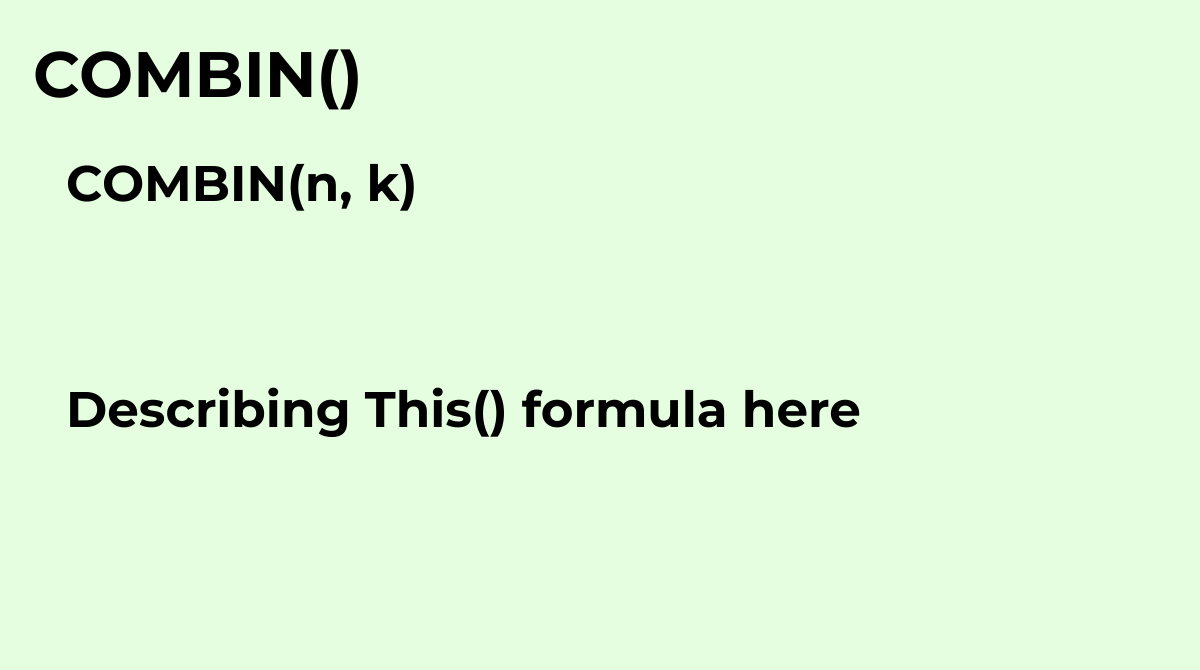Returns the number of ways to choose some number of objects from a pool of a given size of objects.
`COMBIN(n, k)`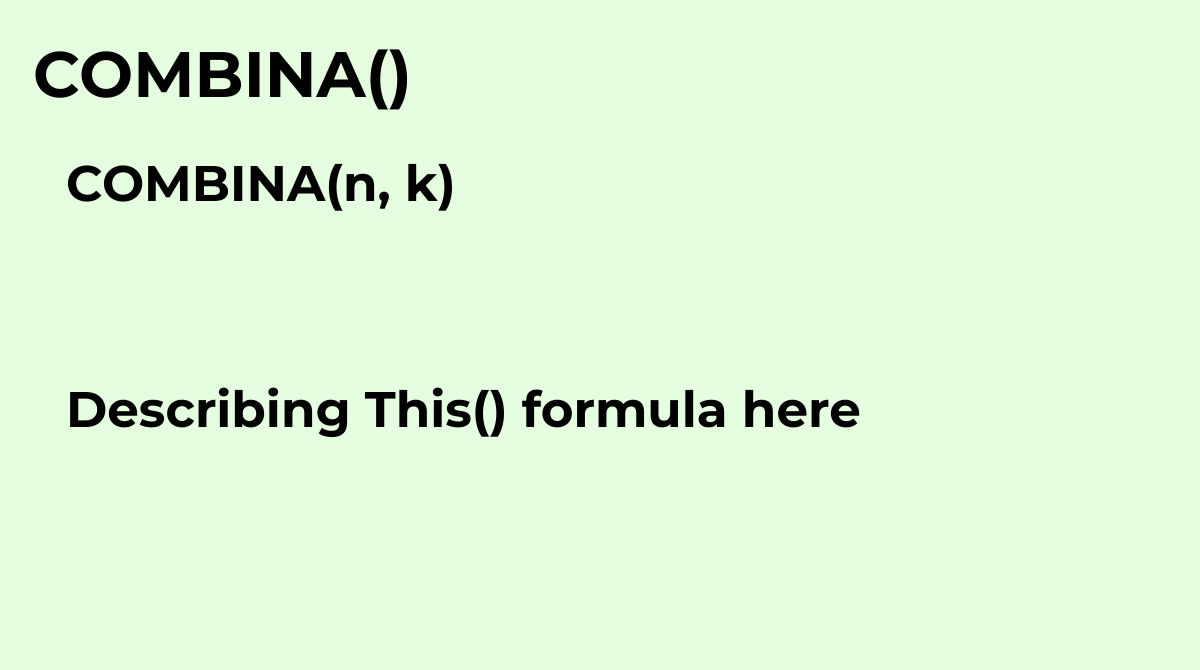Returns the number of ways to choose some number of objects from a pool of a given size of object...
`COMBINA(n, k)`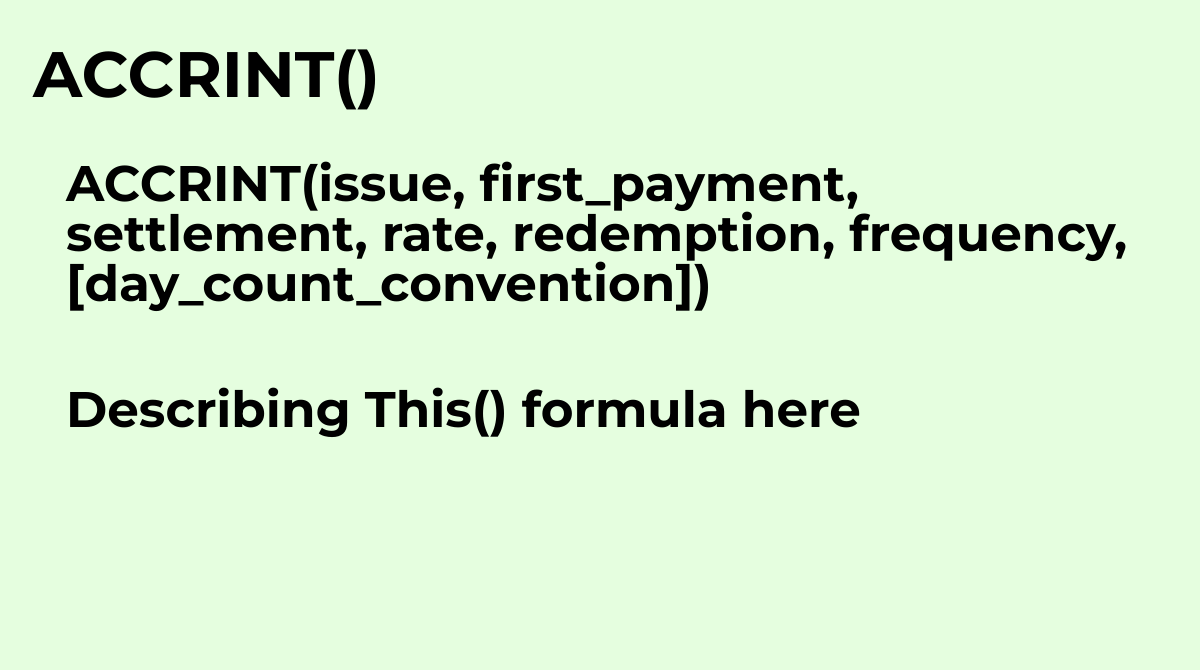Calculates the accrued interest of a security that has periodic payments.Common questions about t...
`ACCRINT(issue, first_payment, settlement, rate, redemption, frequency, [day_count_convention])`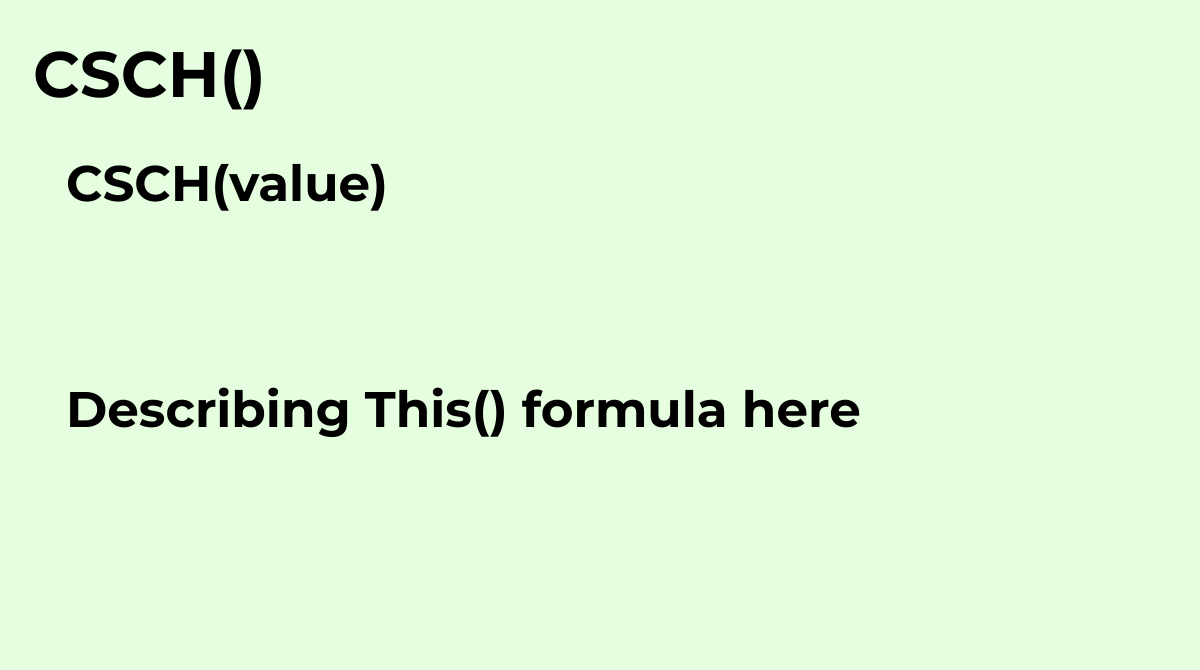The CSCH function returns the hyperbolic cosecant of any real number..
`CSCH(value)`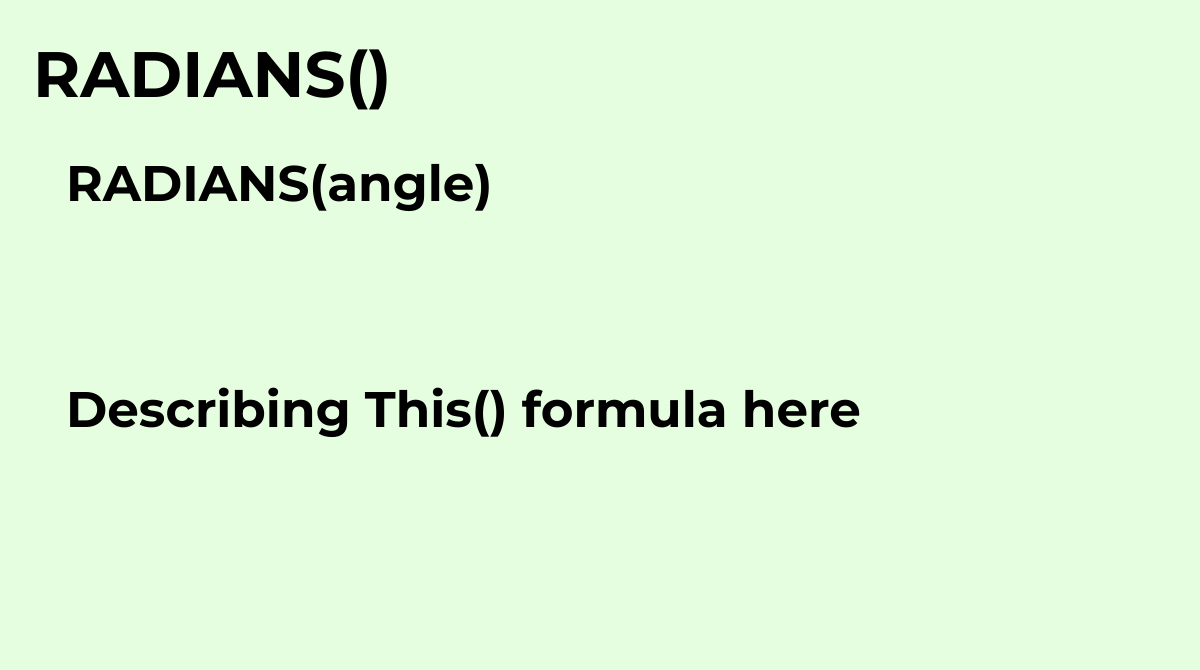Converts an angle value in degrees to radians.
`RADIANS(angle)`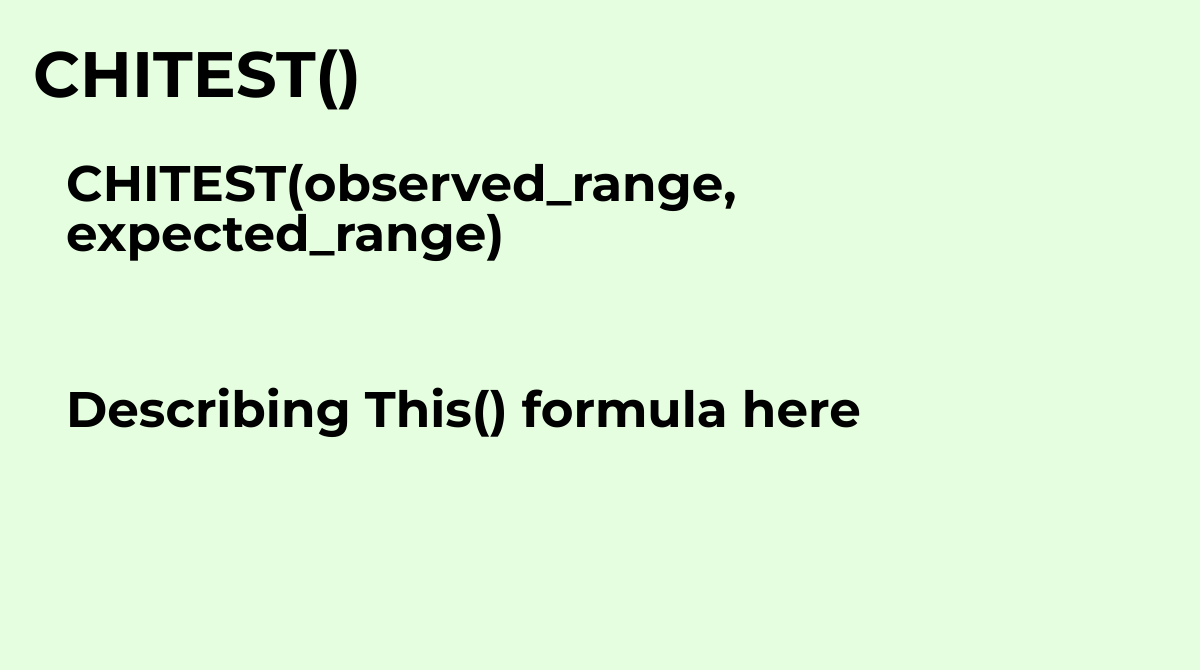Returns the probability associated with a Pearson’s chi-squared test on the two ranges of data. D...
`CHITEST(observed_range, expected_range)`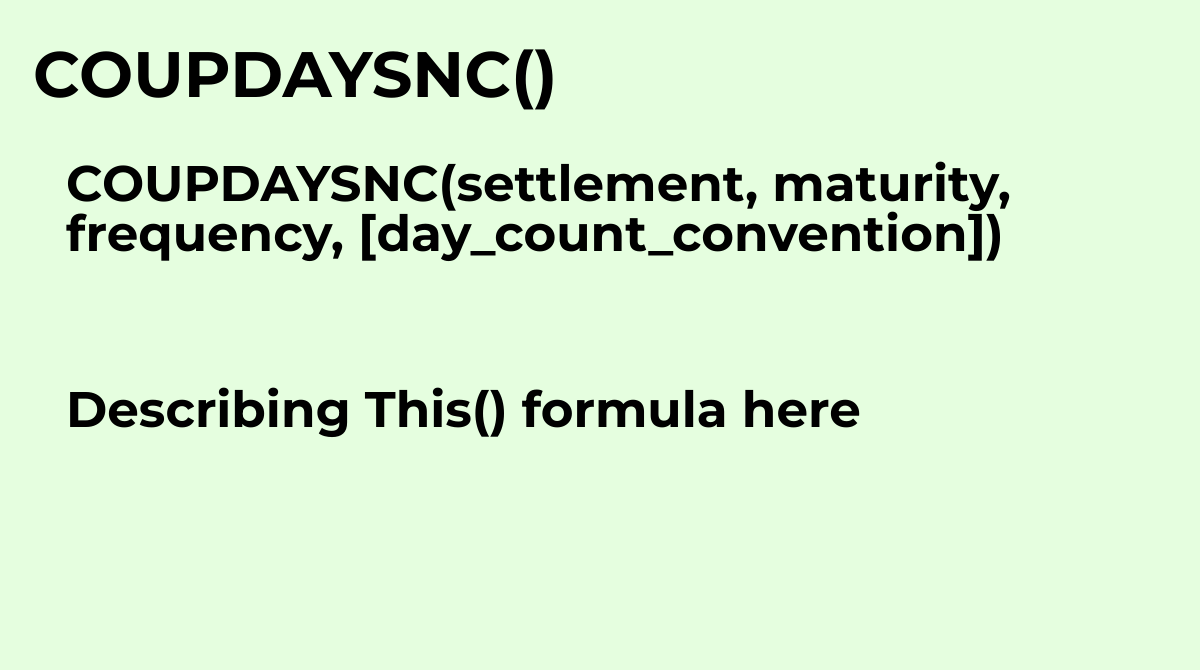Calculates the number of days from the settlement date until the next coupon, or interest payment.
`COUPDAYSNC(settlement, maturity, frequency, [day_count_convention])`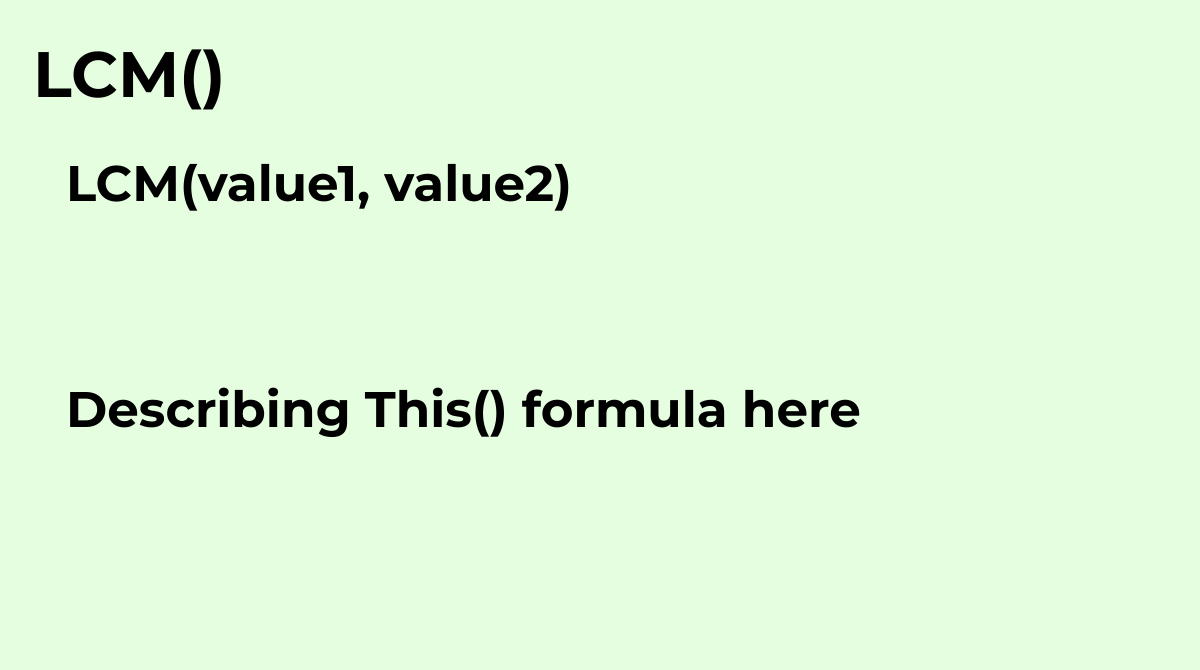Returns the least common multiple of one or more integers.
`LCM(value1, value2)`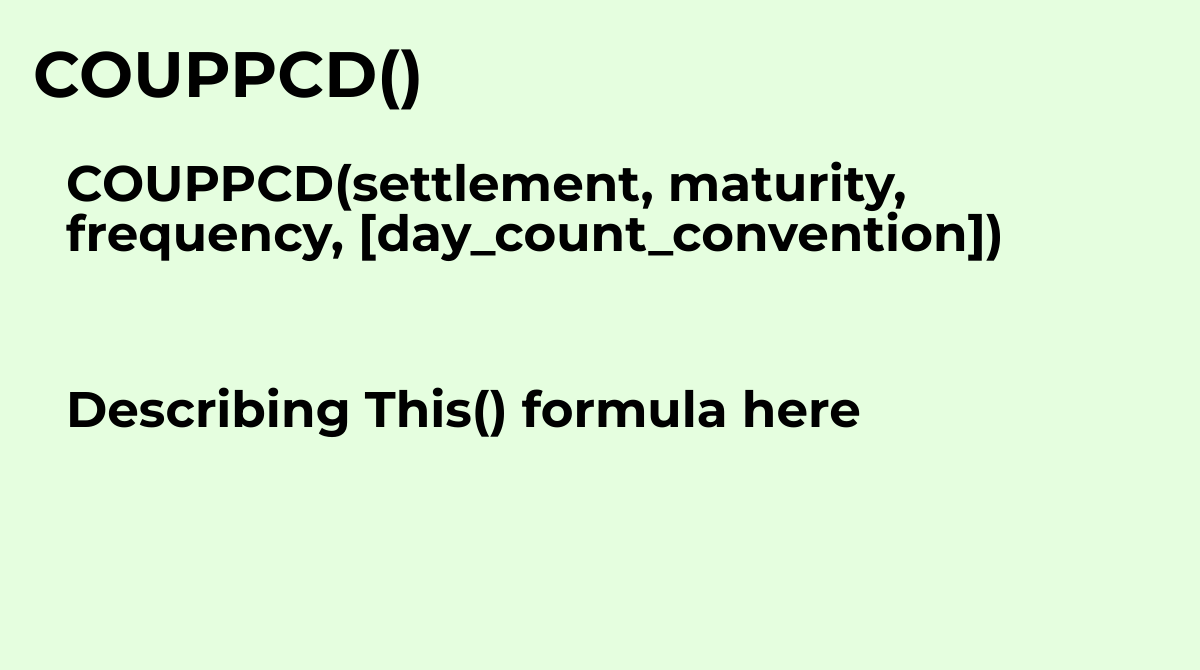Calculates last coupon, or interest payment, date before the settlement date.
`COUPPCD(settlement, maturity, frequency, [day_count_convention])`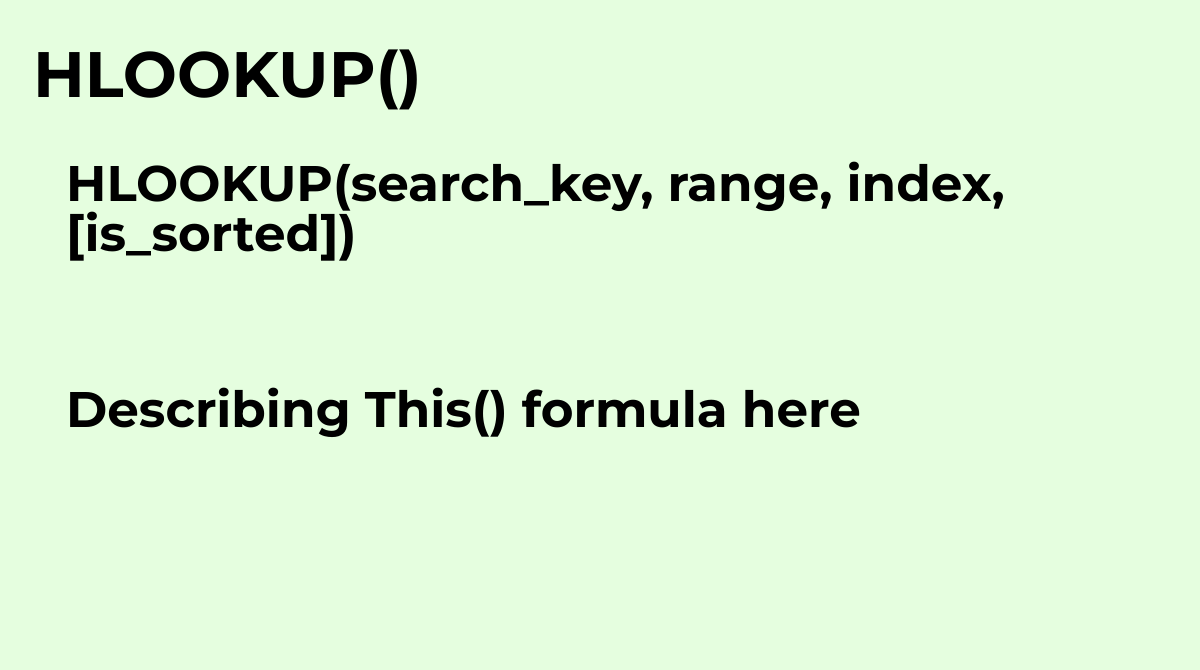Horizontal lookup. Searches across the first row of a range for a key and returns the value of a ...
`HLOOKUP(search_key, range, index, [is_sorted])`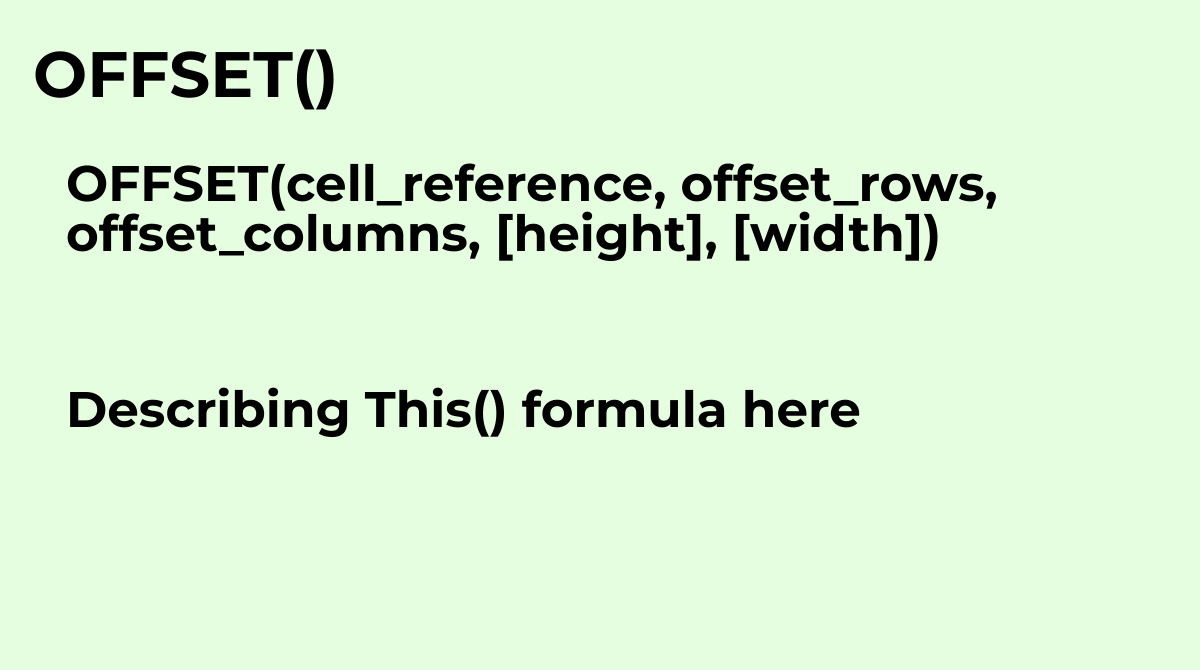Returns a range reference shifted a specified number of rows and columns from a starting cell ref...
`OFFSET(cell_reference, offset_rows, offset_columns, [height], [width])`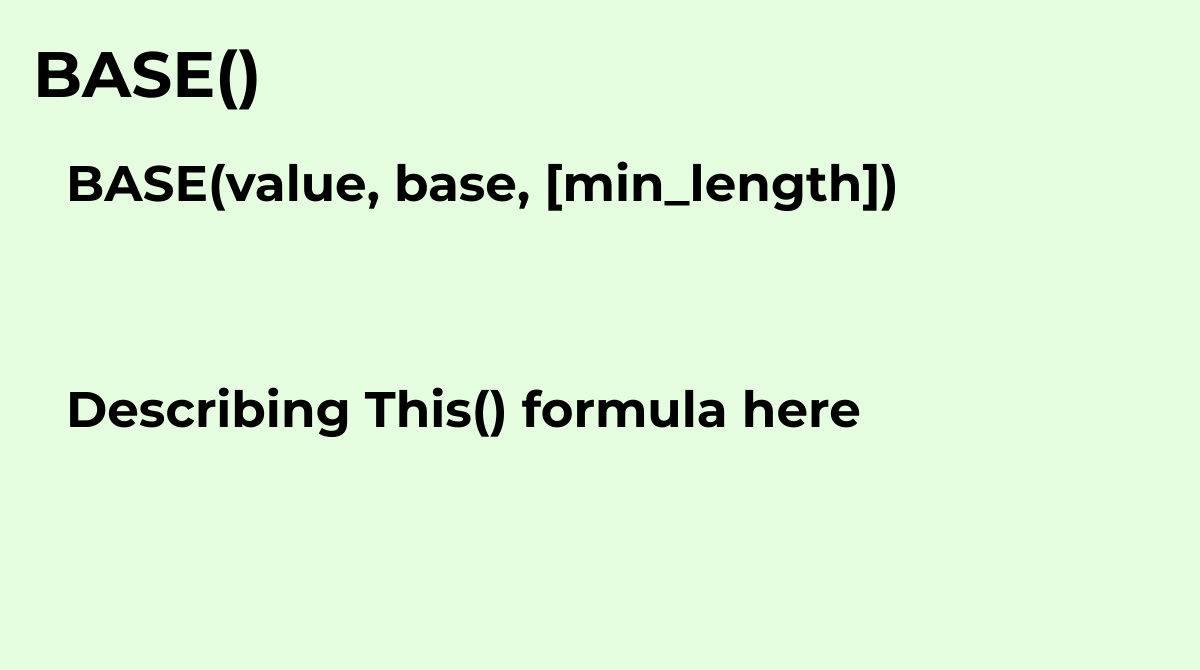Converts a number into a text representation in another base, for example, base 2 for binary.The ...
`BASE(value, base, [min_length])`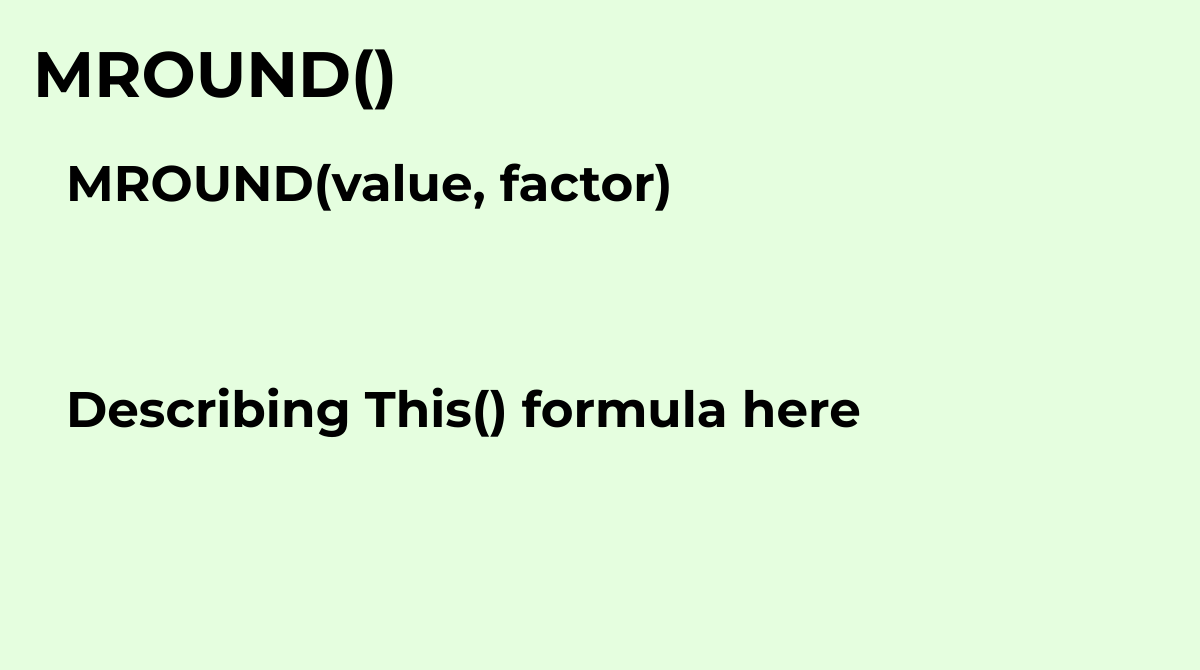Rounds one number to the nearest integer multiple of another.
`MROUND(value, factor)`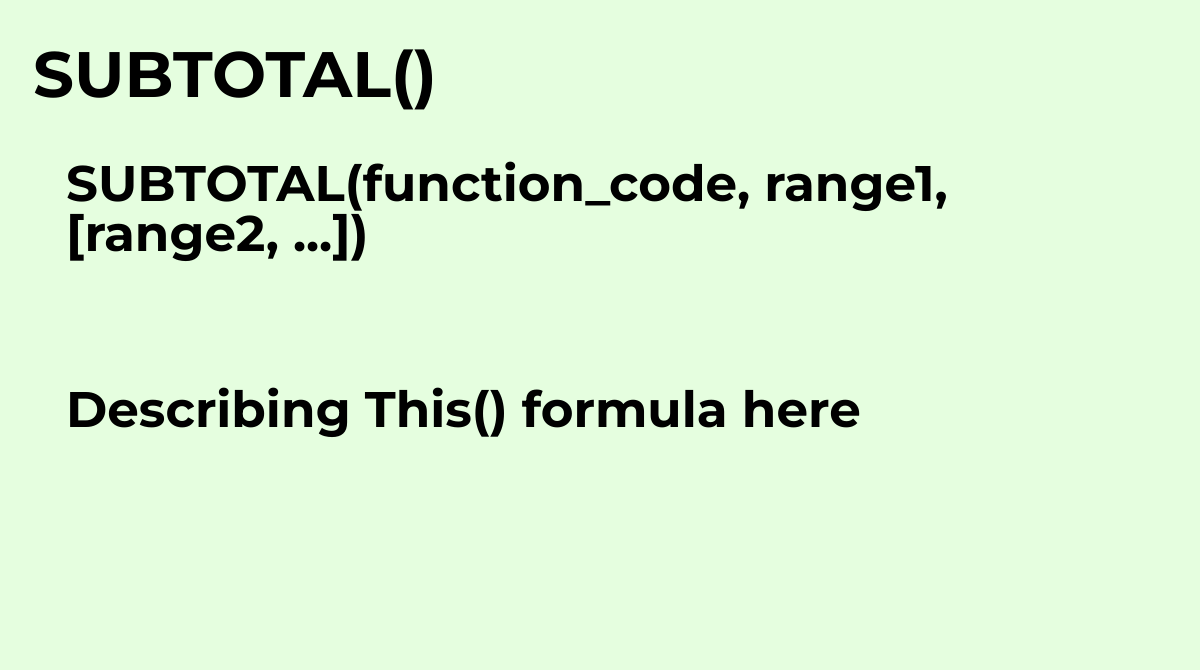Returns a subtotal for a vertical range of cells using a specified aggregation function.
`SUBTOTAL(function_code, range1, [range2, ...])`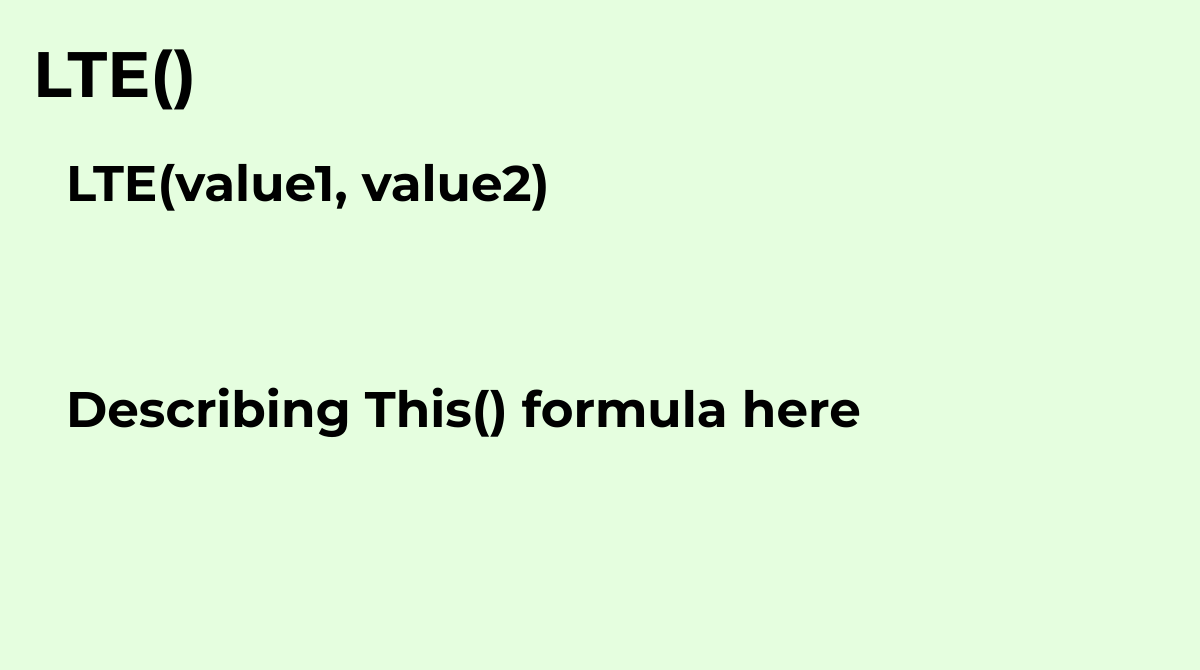Returns `TRUE` if the first argument is less than or equal to the second, and `FALSE` otherwise. ...
`LTE(value1, value2)`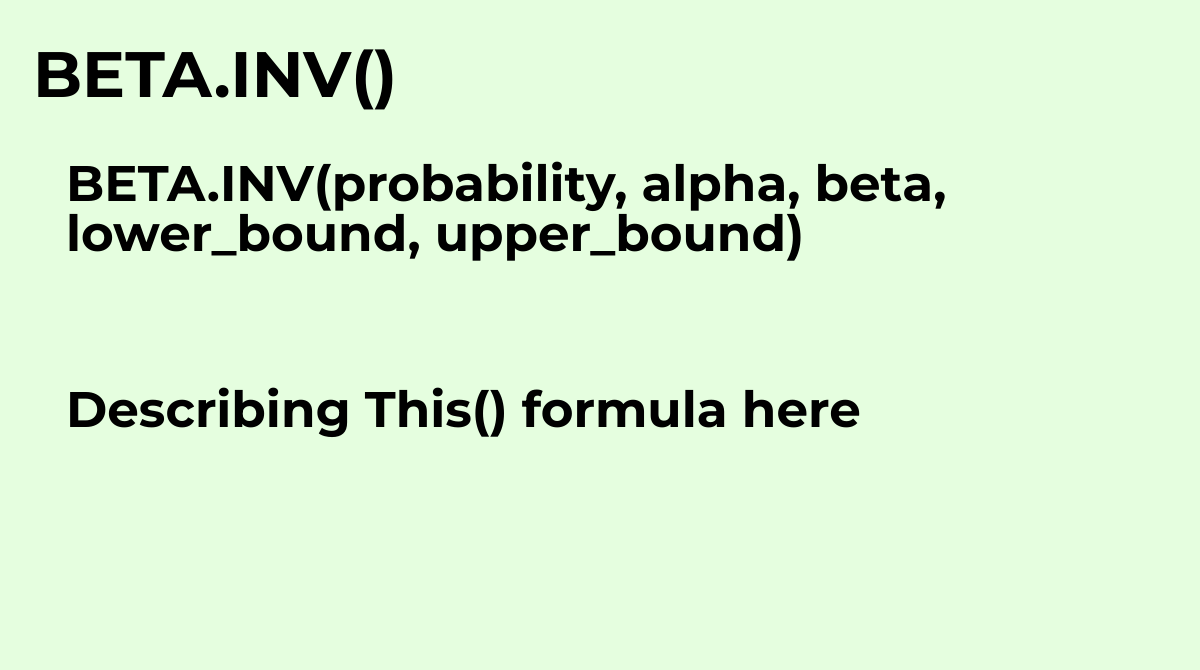Returns the value of the inverse beta distribution function for a given probability..
`BETA.INV(probability, alpha, beta, lower_bound, upper_bound)`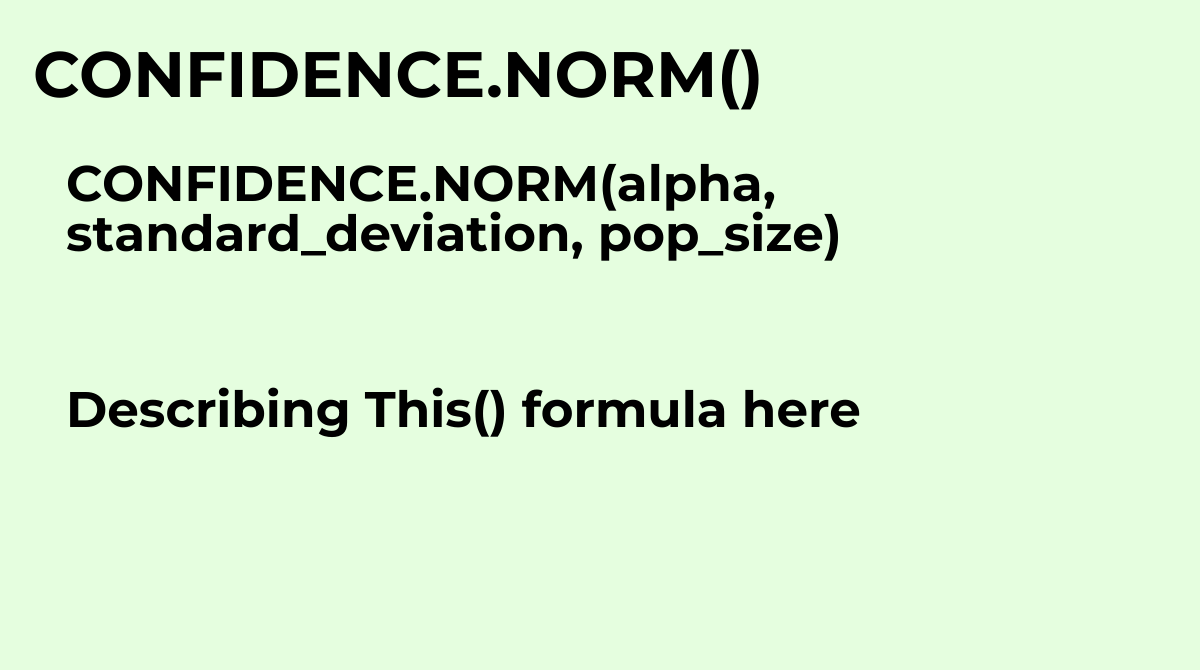Calculates the width of half the confidence interval for a normal distribution..
`CONFIDENCE.NORM(alpha, standard_deviation, pop_size)`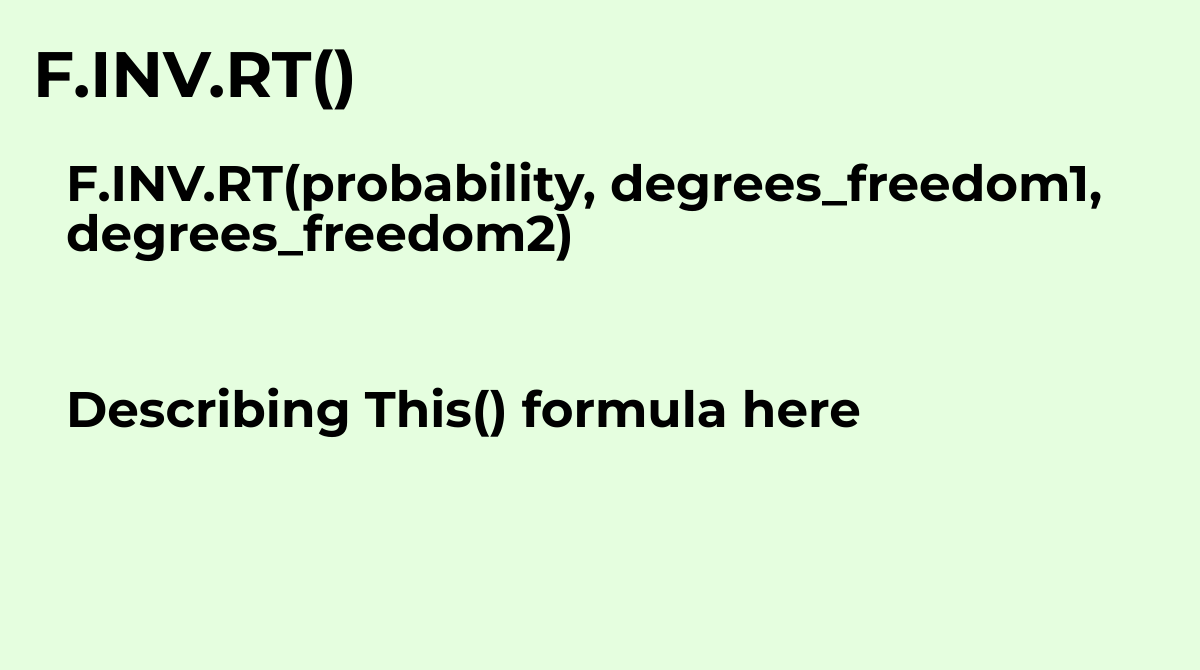Calculates the inverse of the right-tailed F probability distribution. Also called the Fisher-Sne...
`F.INV.RT(probability, degrees_freedom1, degrees_freedom2)`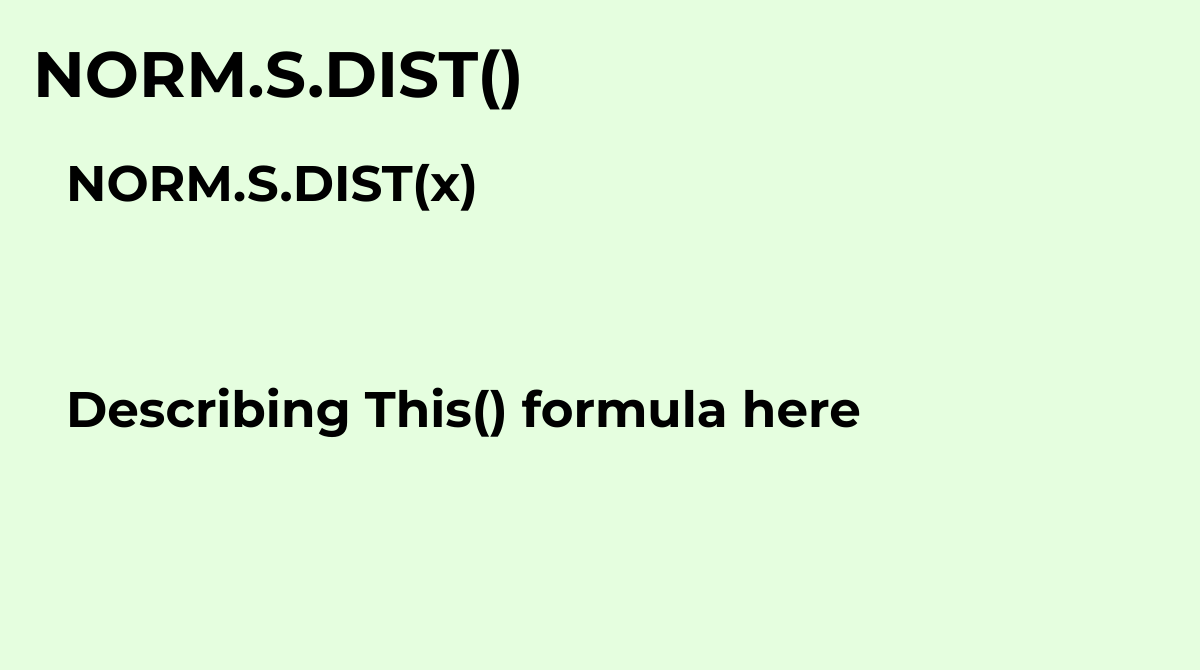See NORMSDIST
`NORM.S.DIST(x)`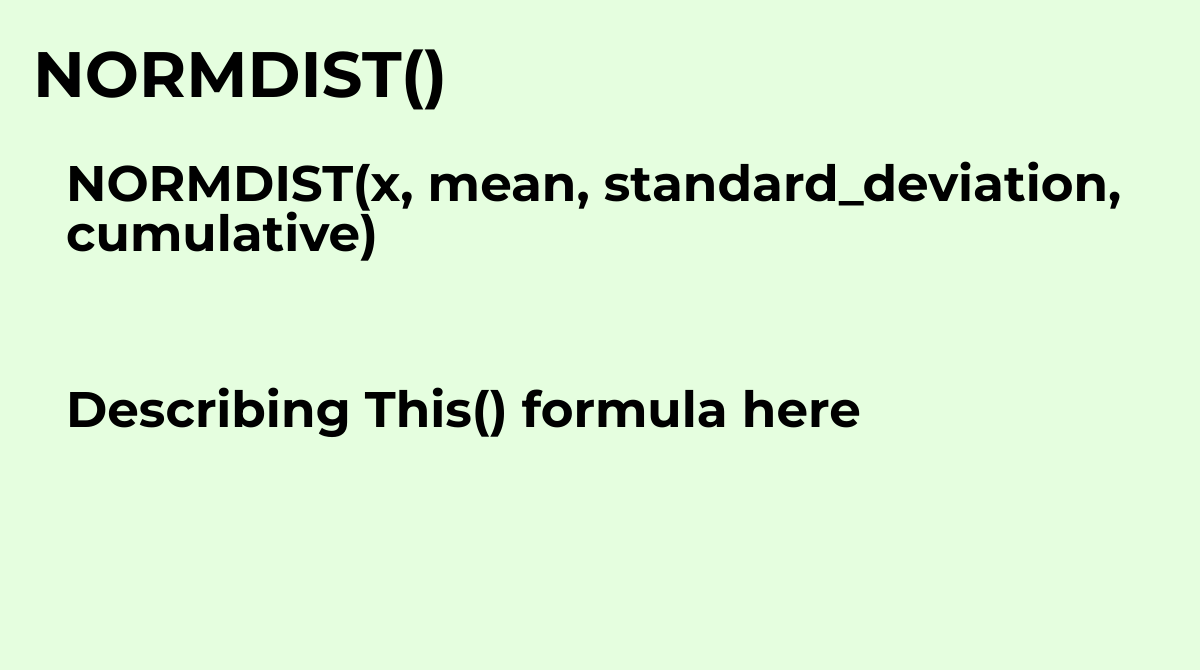Returns the value of the normal distribution function (or normal cumulative distribution function...
`NORMDIST(x, mean, standard_deviation, cumulative)`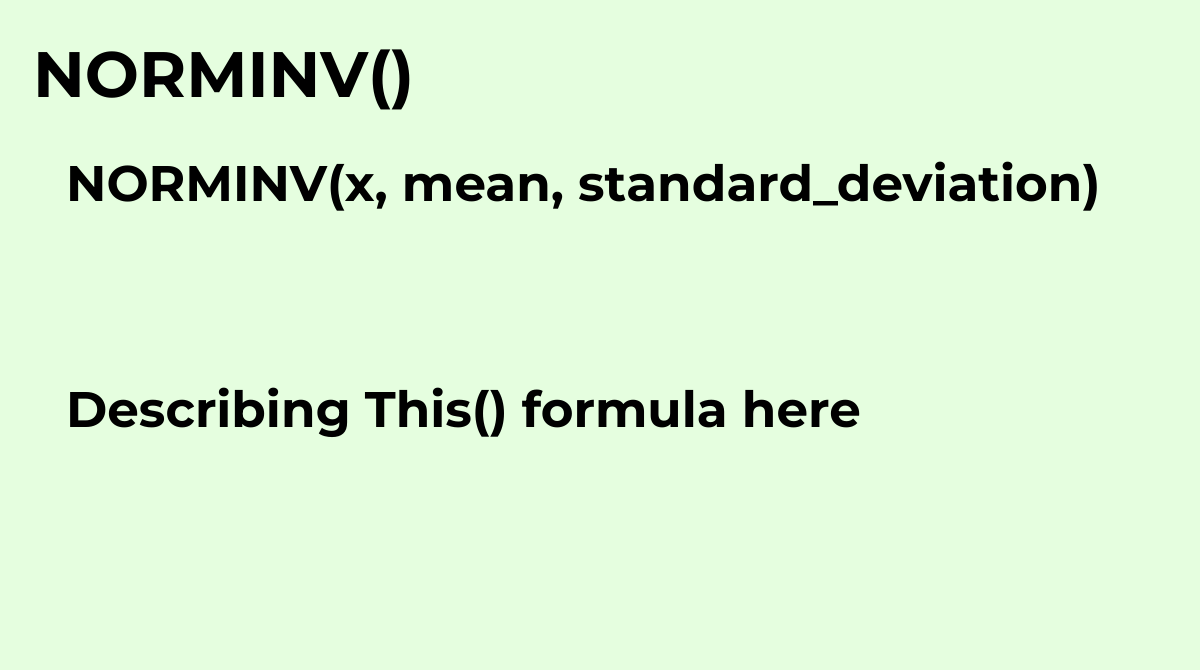Returns the value of the inverse normal distribution function for a specified value, mean, and st...
`NORMINV(x, mean, standard_deviation)`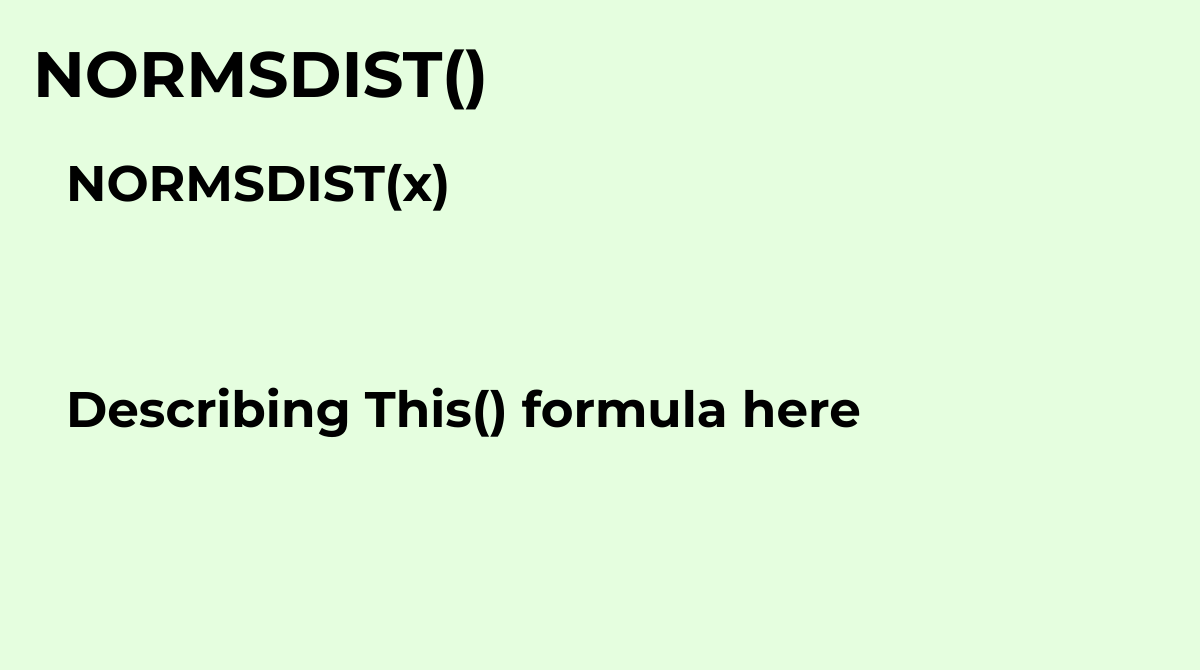Returns the value of the standard normal cumulative distribution function for a specified value.
`NORMSDIST(x)`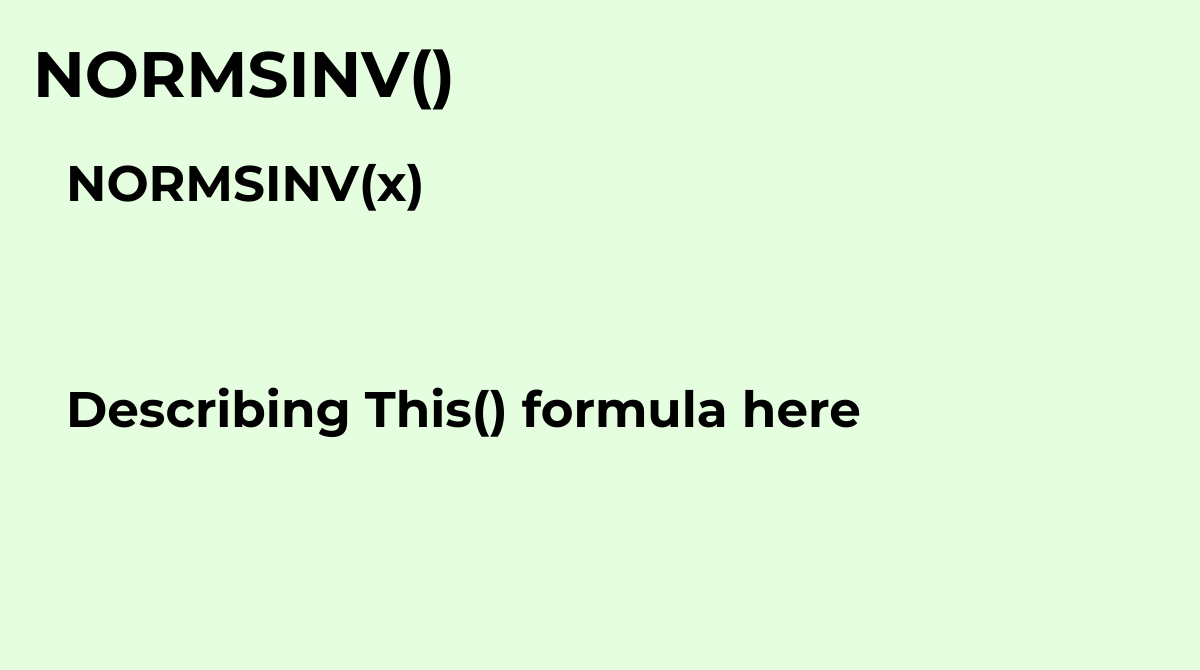Returns the value of the inverse standard normal distribution function for a specified value.
`NORMSINV(x)`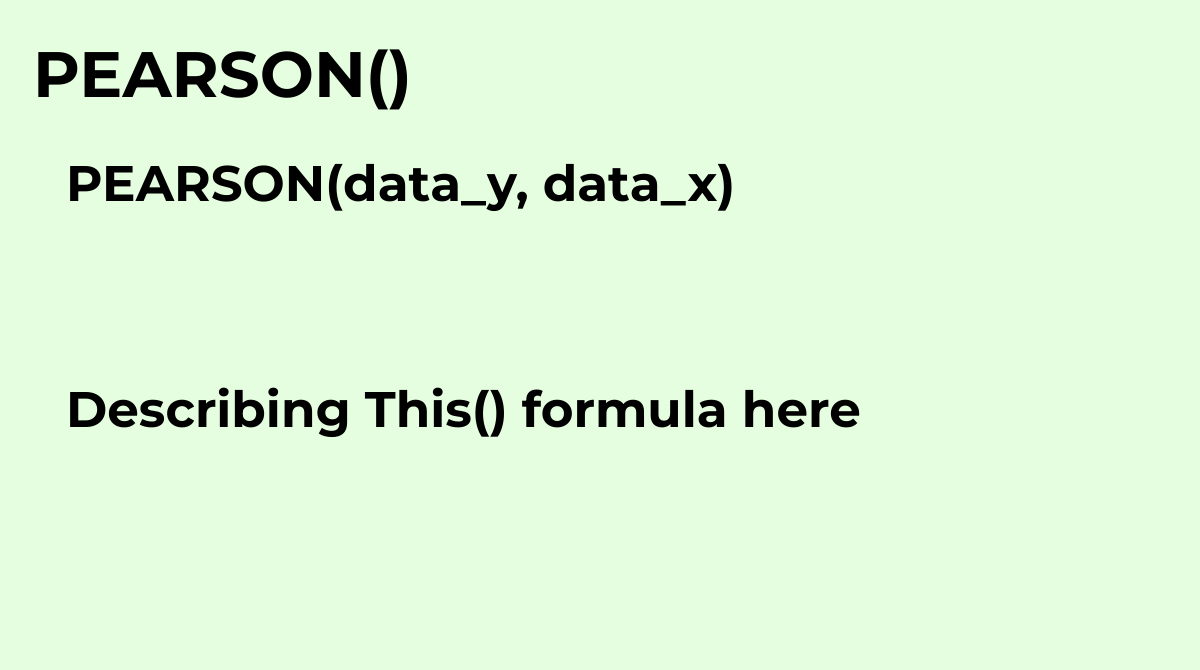Calculates r, the Pearson product-moment correlation coefficient of a dataset.
`PEARSON(data_y, data_x)`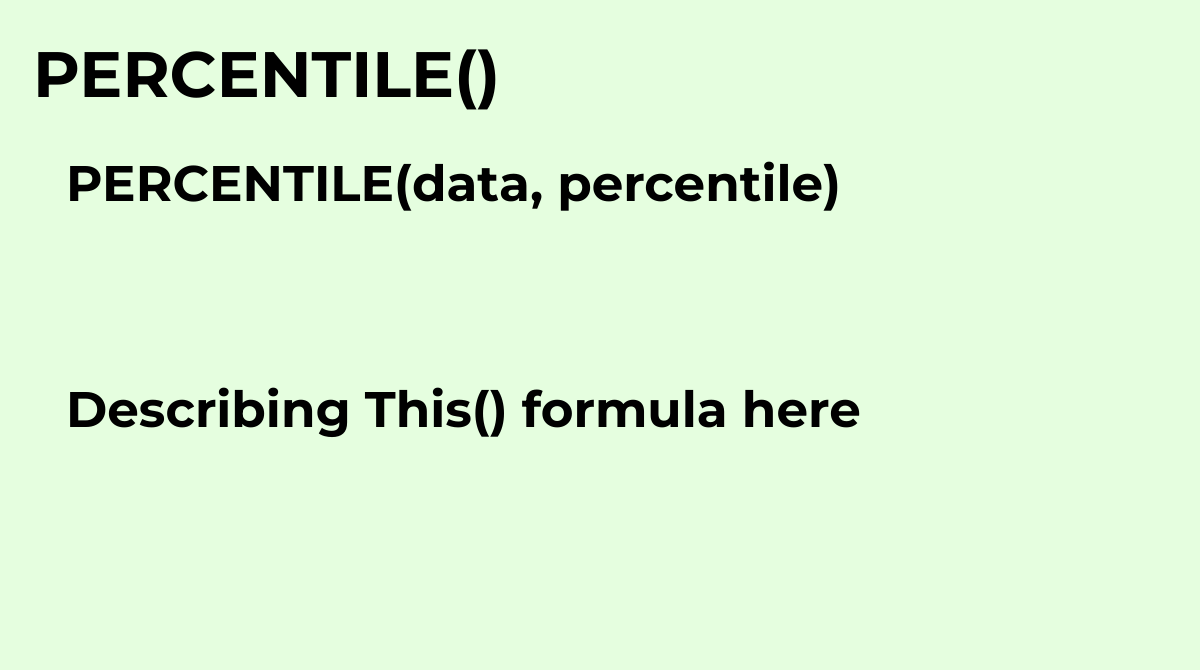Returns the value at a given percentile of a dataset.Common questions about the PERCENTILE formul...
`PERCENTILE(data, percentile)`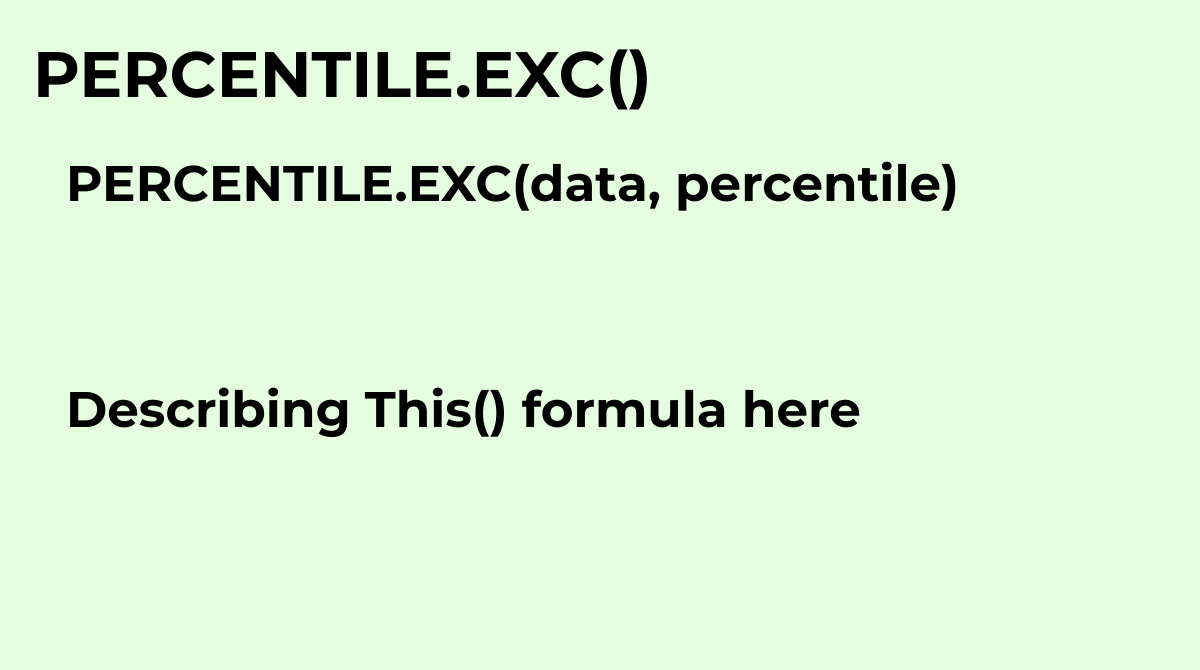Returns the value at a given percentile of a dataset, exclusive of 0 and 1..
`PERCENTILE.EXC(data, percentile)`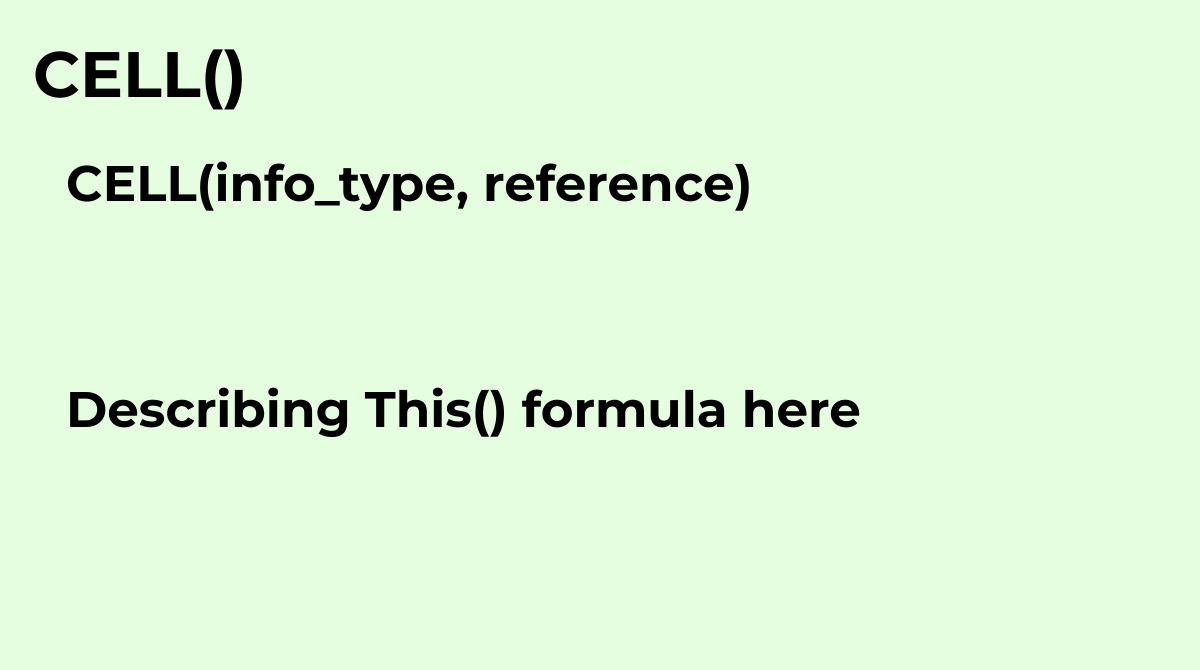Returns the requested information about the specified cell.
`CELL(info_type, reference)`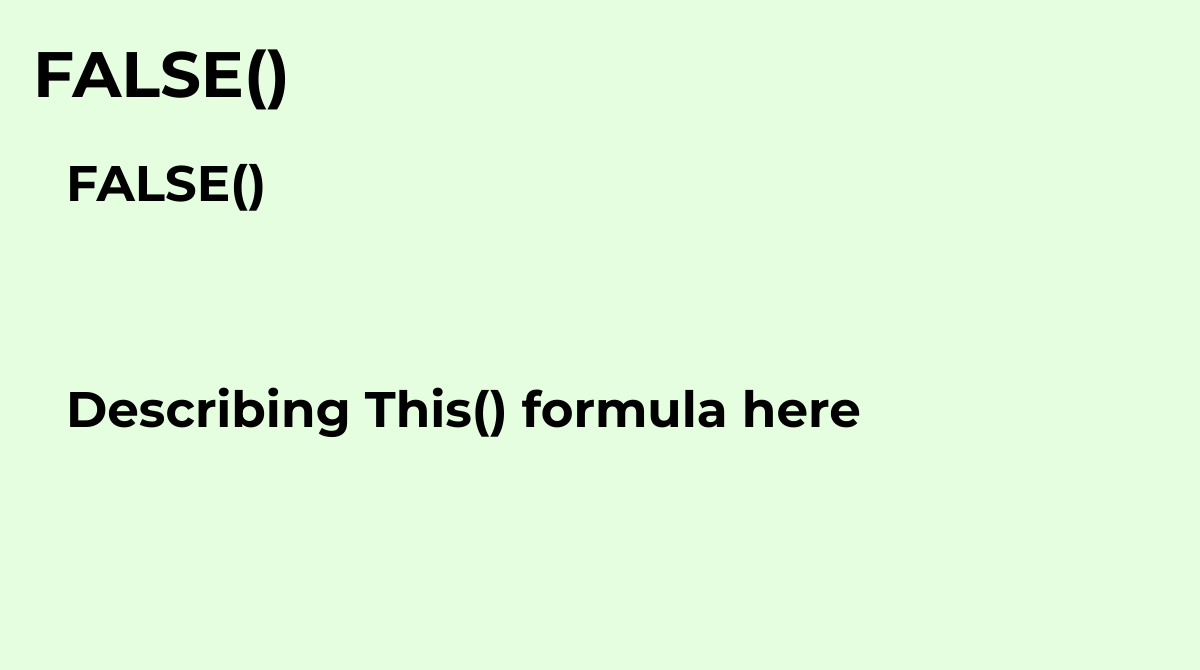Returns the logical value `FALSE`.
`FALSE()`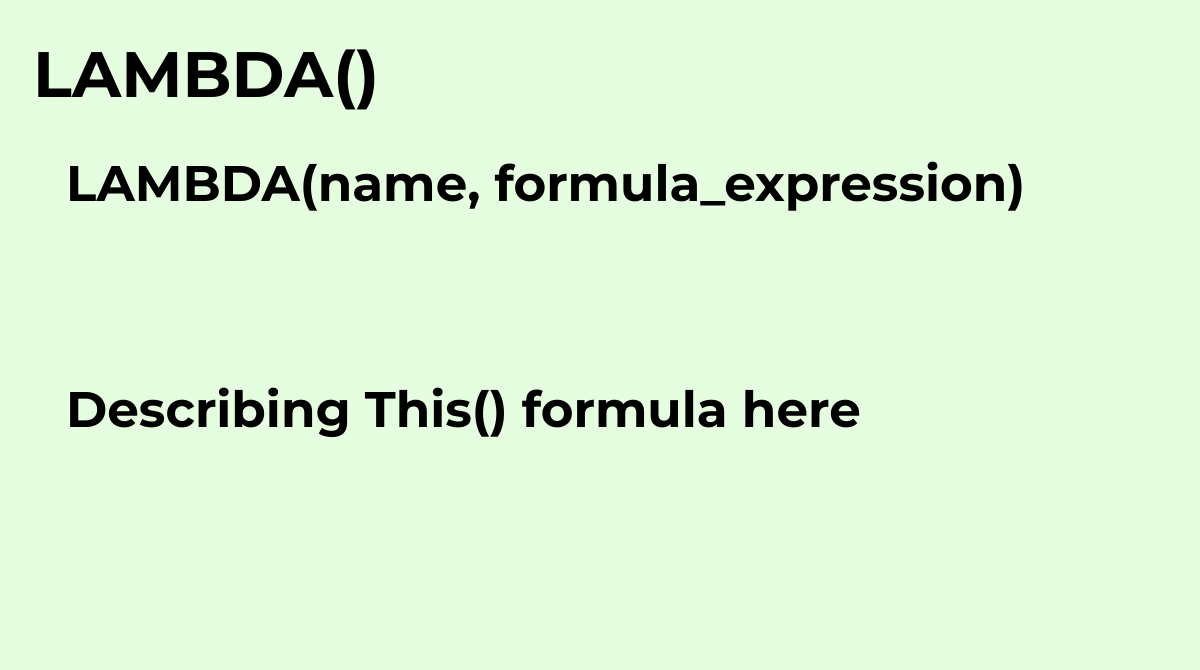Creates and returns a custom function with a set of names and a formula_expression that uses them...
`LAMBDA(name, formula_expression)`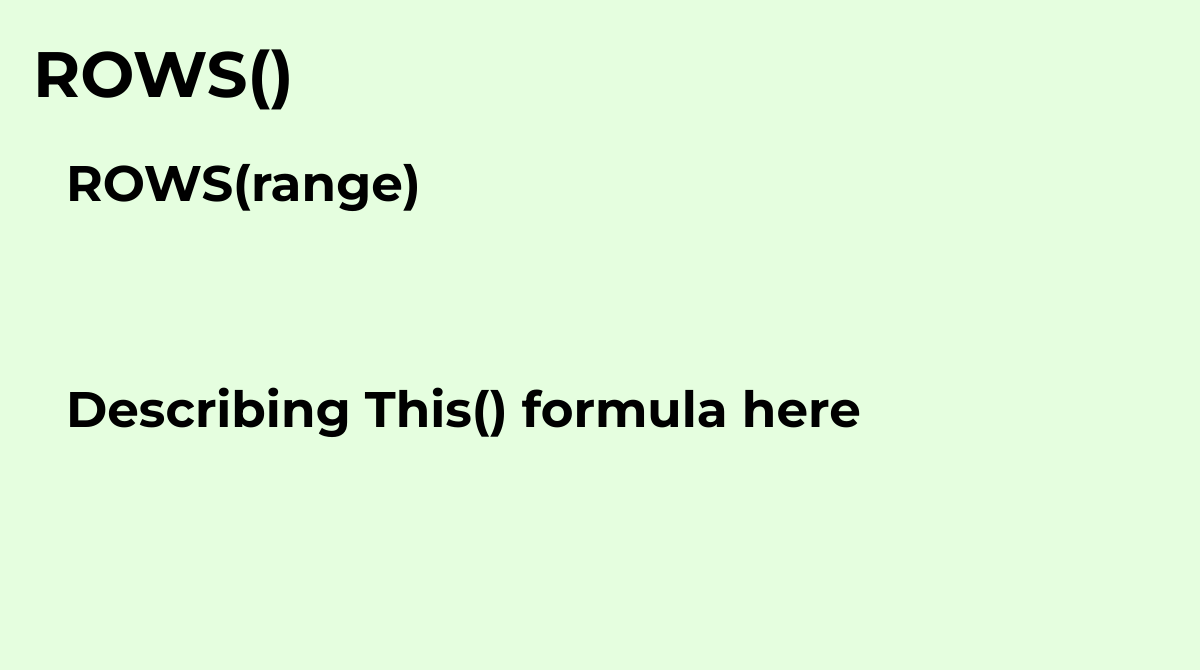Returns the number of rows in a specified array or range.
`ROWS(range)`Returns the values in the result range based on the position where a match was found in the looku...
`XLOOKUP(search_key, lookup_range, result_range, missing_value, [match_mode], [search_mode])`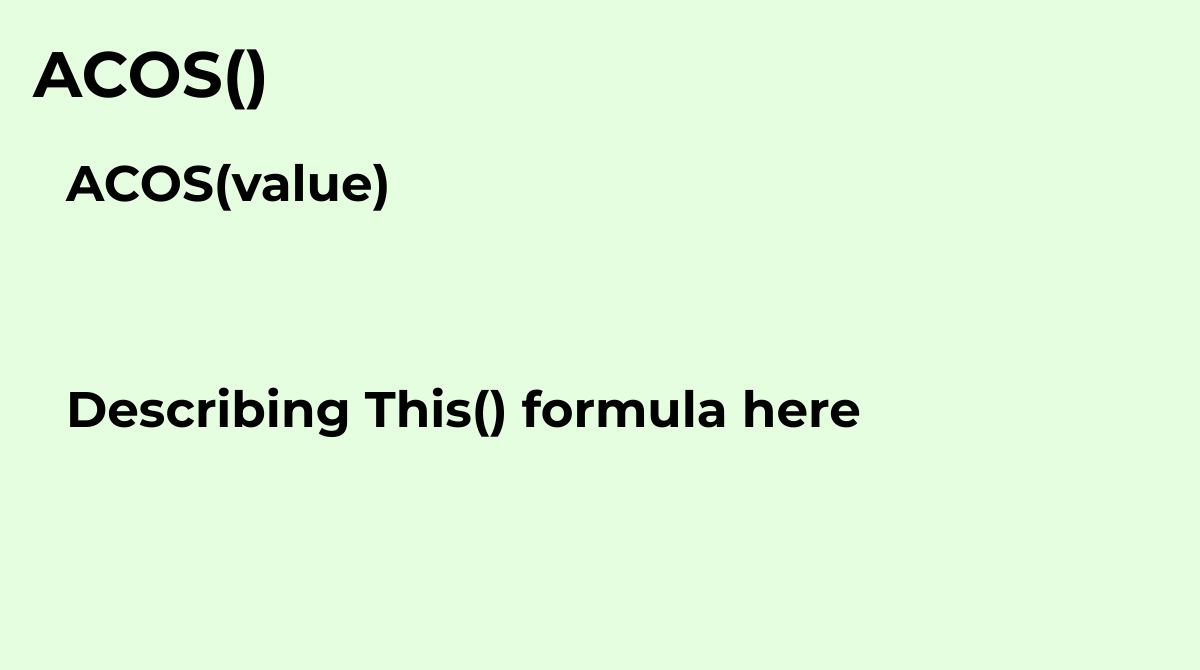Returns the inverse cosine of a value, in radians.
`ACOS(value)`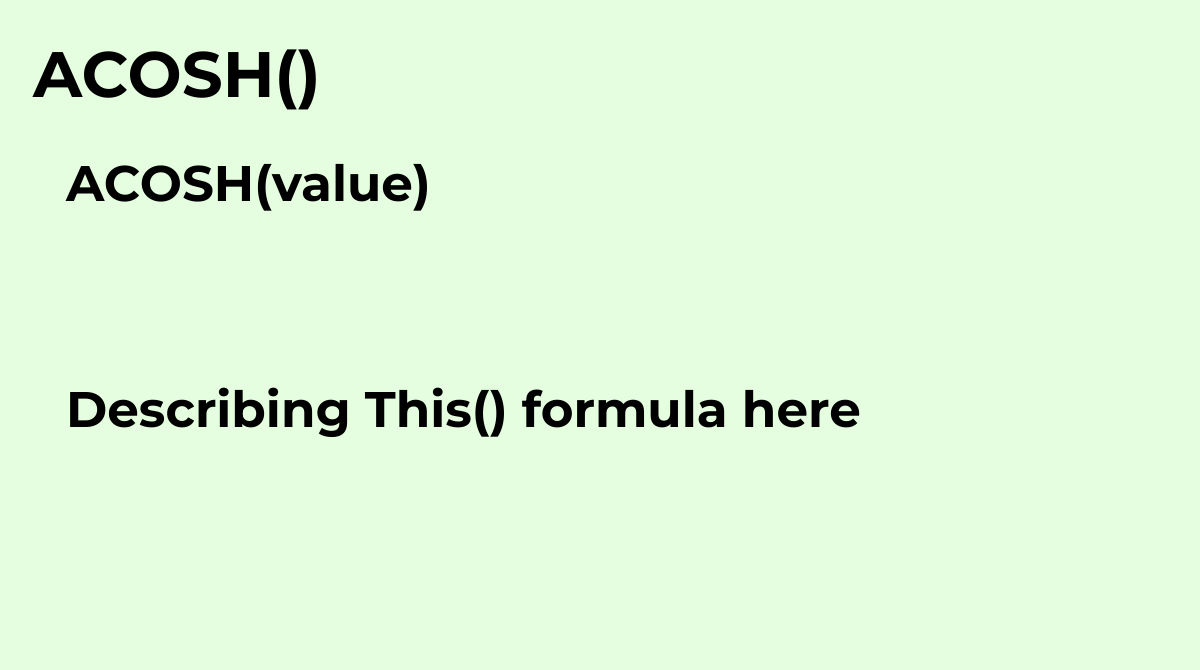Returns the inverse hyperbolic cosine of a number.
`ACOSH(value)`Returns the inverse cotangent of a value, in radians..
`ACOT(value)`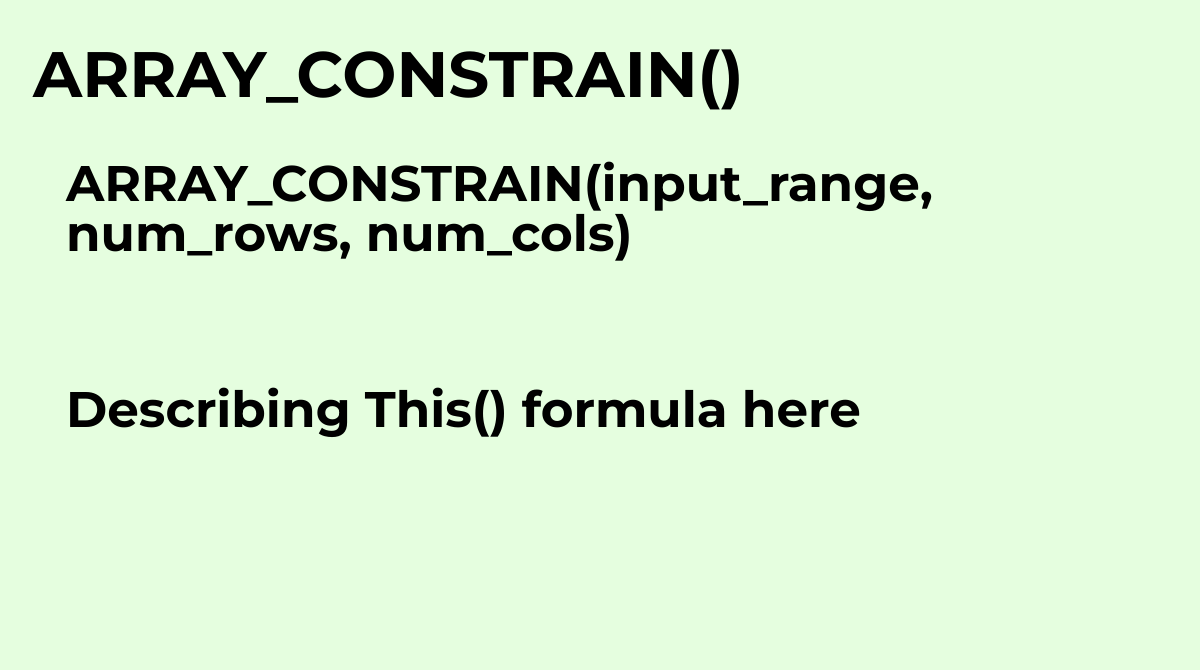Constrains an array result to a specified size.Common Questions about the ARRAY_CONSTRAIN Formula...
`ARRAY_CONSTRAIN(input_range, num_rows, num_cols)`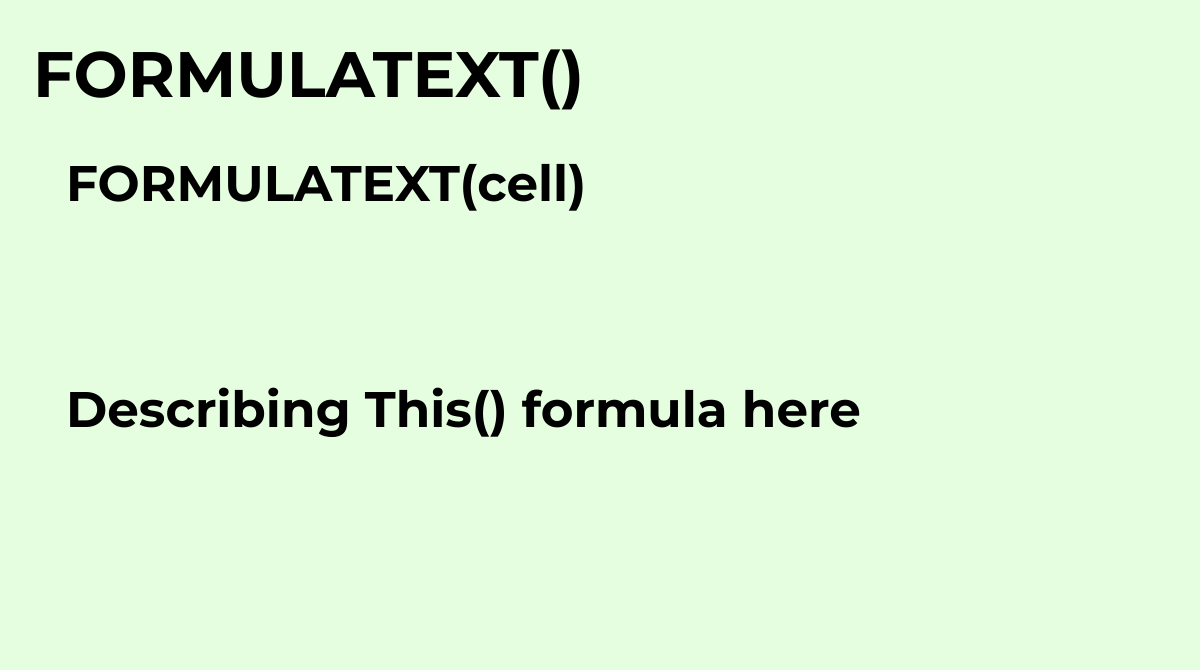Returns the formula as a string.What are the common questions about the FORMULATEXT formula?The c...
`FORMULATEXT(cell)`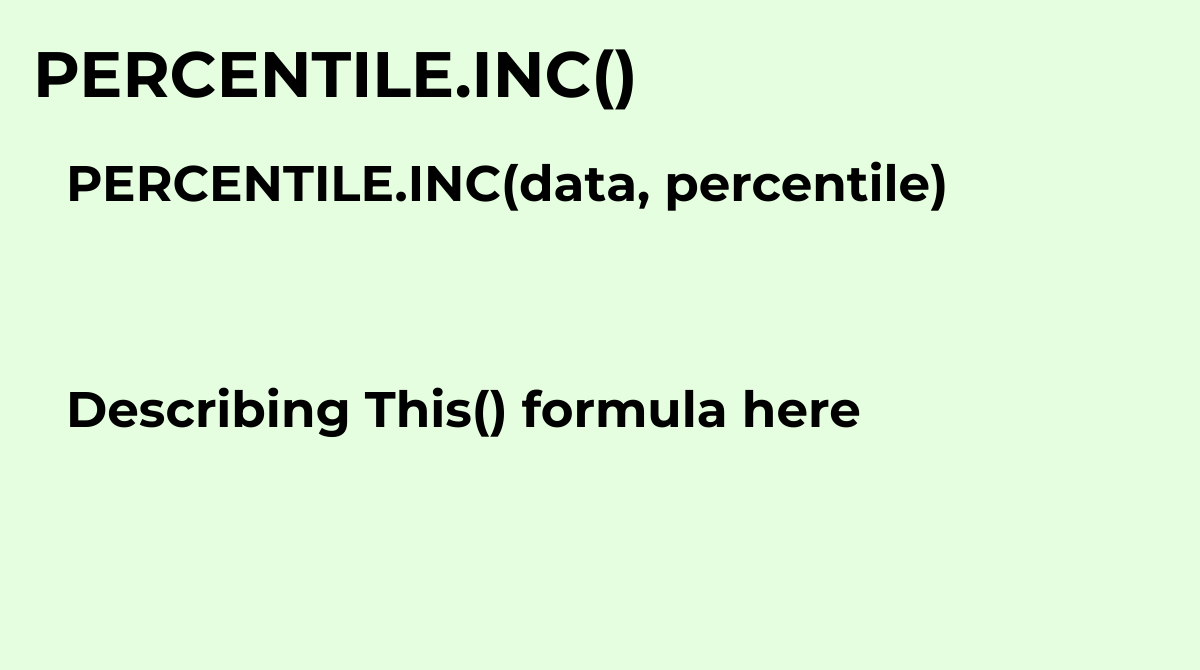See PERCENTILE
`PERCENTILE.INC(data, percentile)`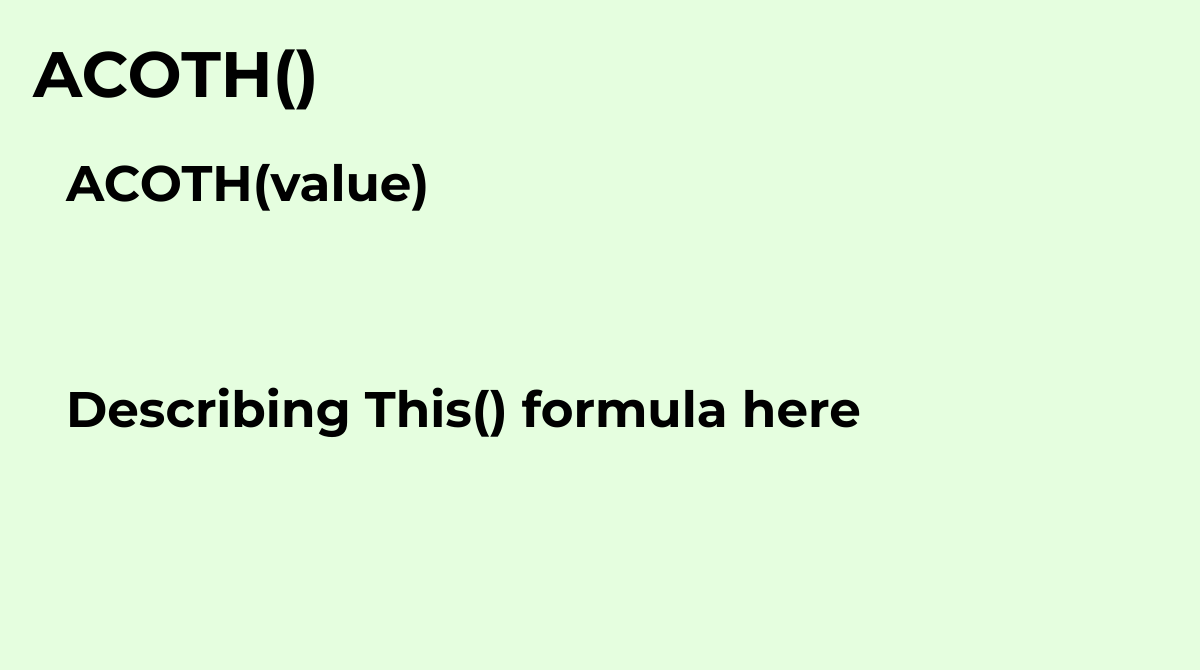Returns the inverse hyperbolic cotangent of a value, in radians. Must not be between -1 and 1, in...
`ACOTH(value)`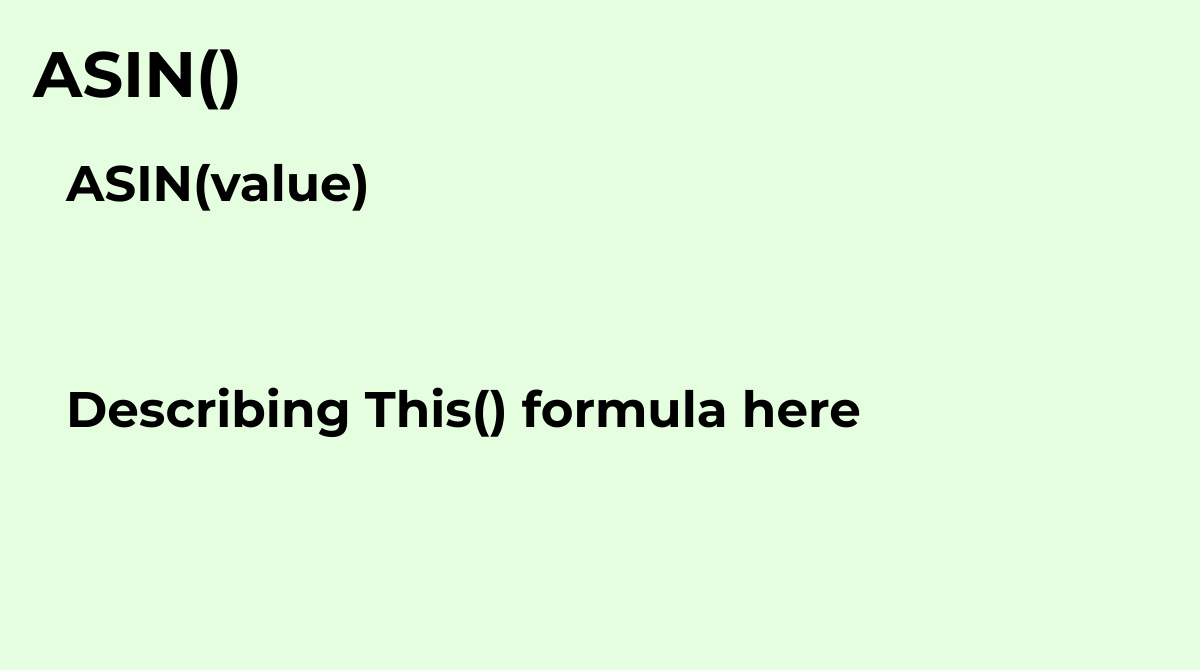Returns the inverse sine of a value, in radians.
`ASIN(value)`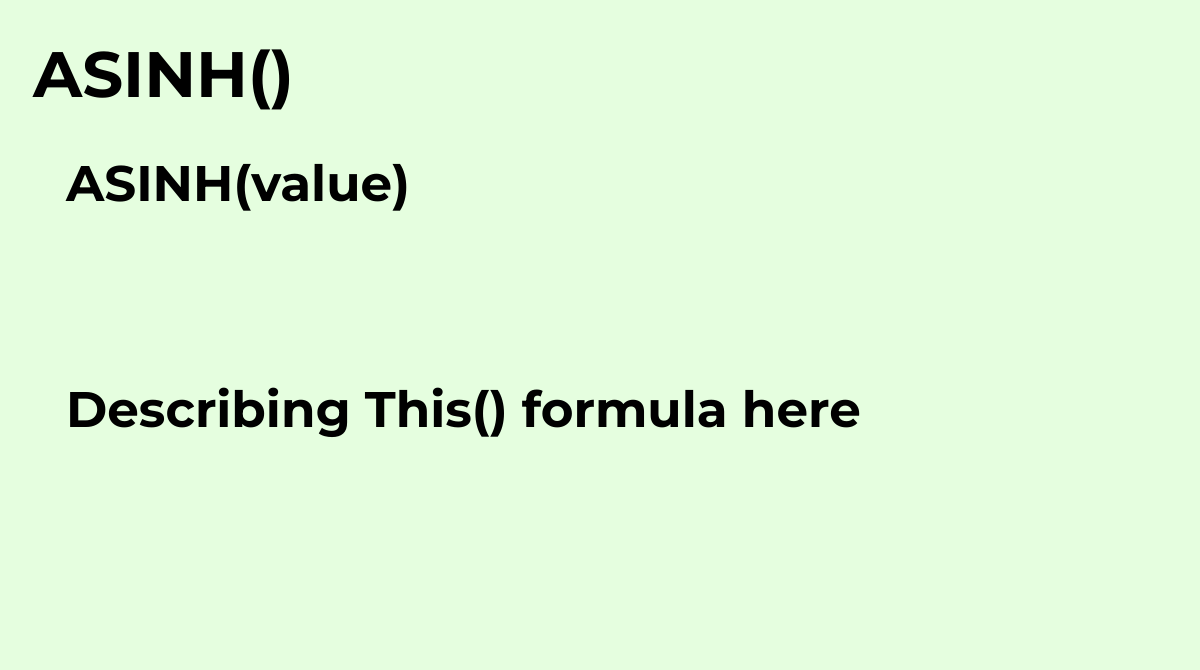Returns the inverse hyperbolic sine of a number.
`ASINH(value)`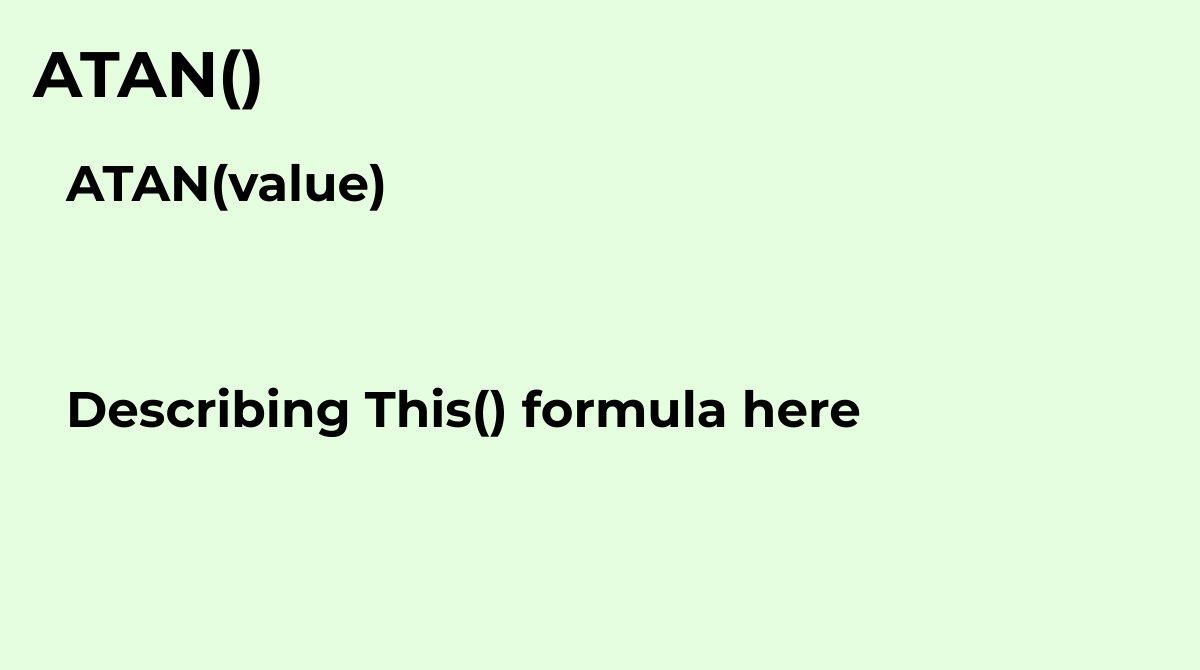Returns the inverse tangent of a value, in radians.
`ATAN(value)`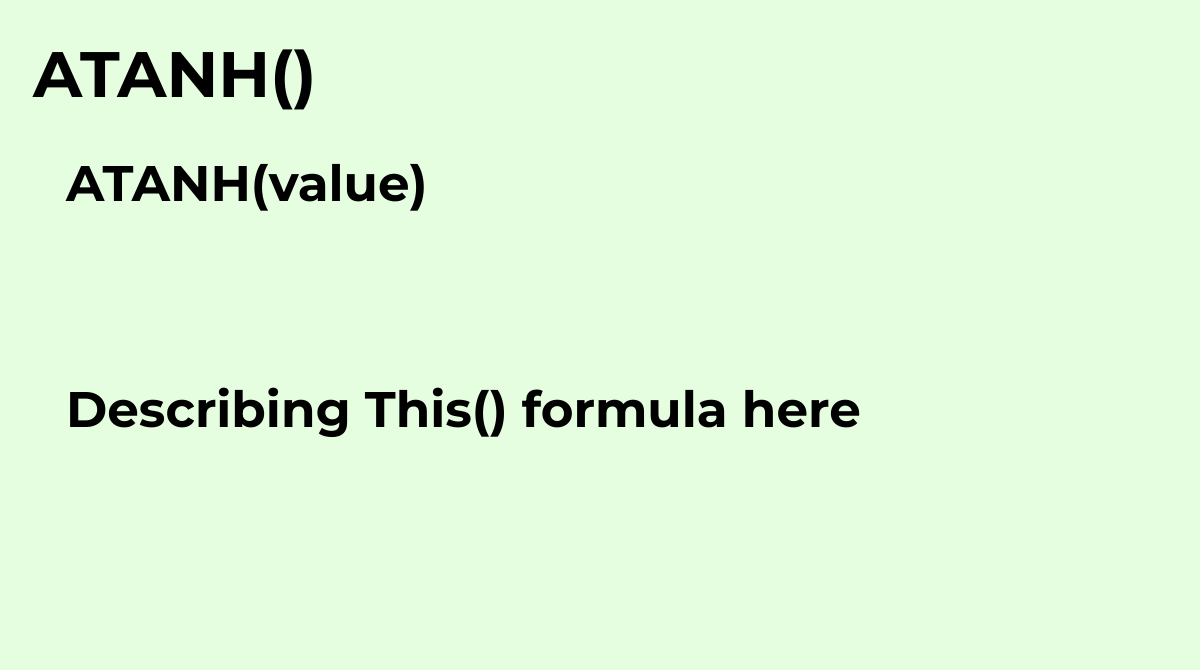Returns the inverse hyperbolic tangent of a number.
`ATANH(value)`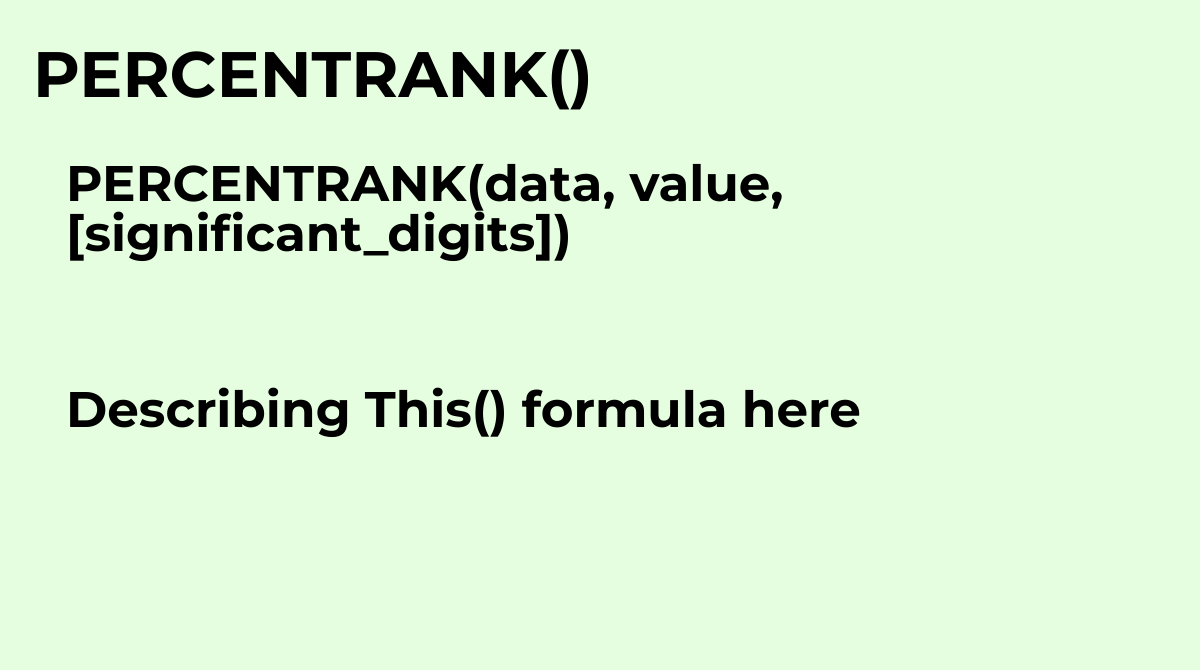Returns the percentage rank (percentile) of a specified value in a dataset.Common questions about...
`PERCENTRANK(data, value, [significant_digits])`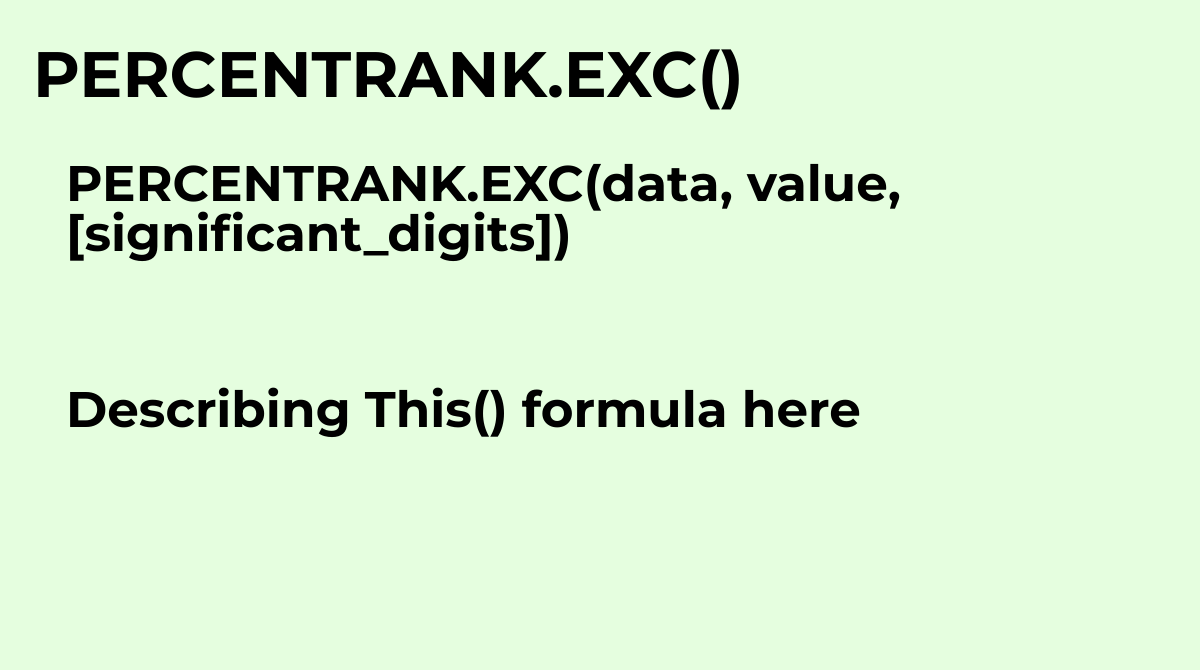Returns the percentage rank (percentile) from 0 to 1 exclusive of a specified value in a dataset.
`PERCENTRANK.EXC(data, value, [significant_digits])`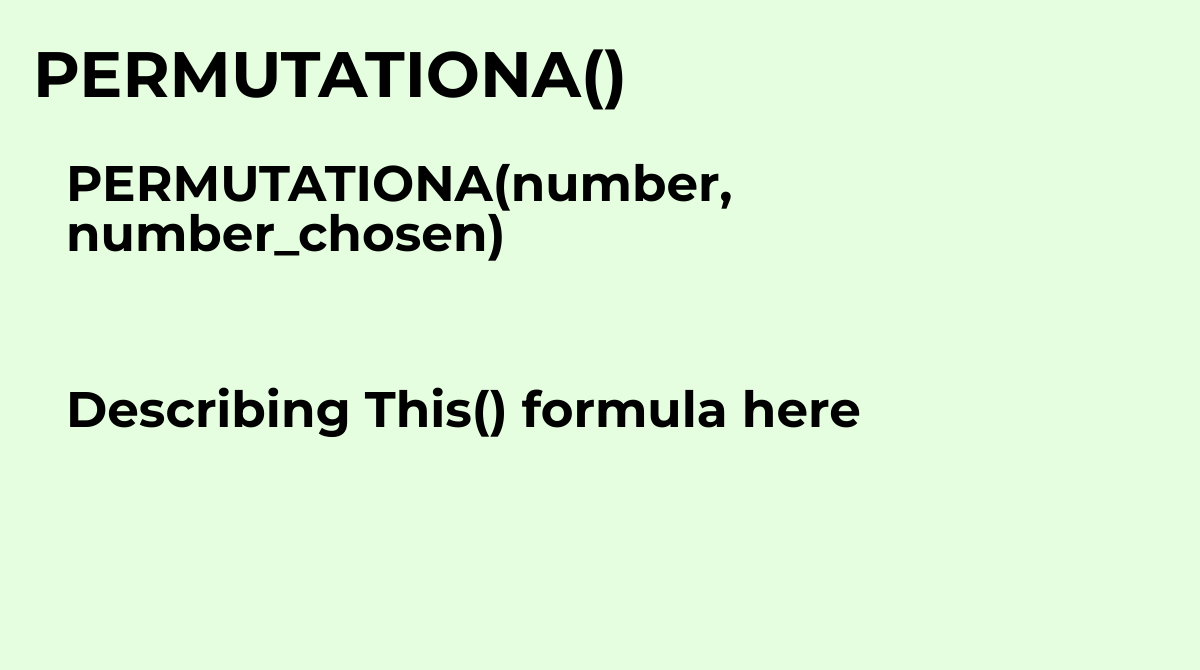Returns the number of permutations for selecting a group of objects (with replacement) from a tot...
`PERMUTATIONA(number, number_chosen)`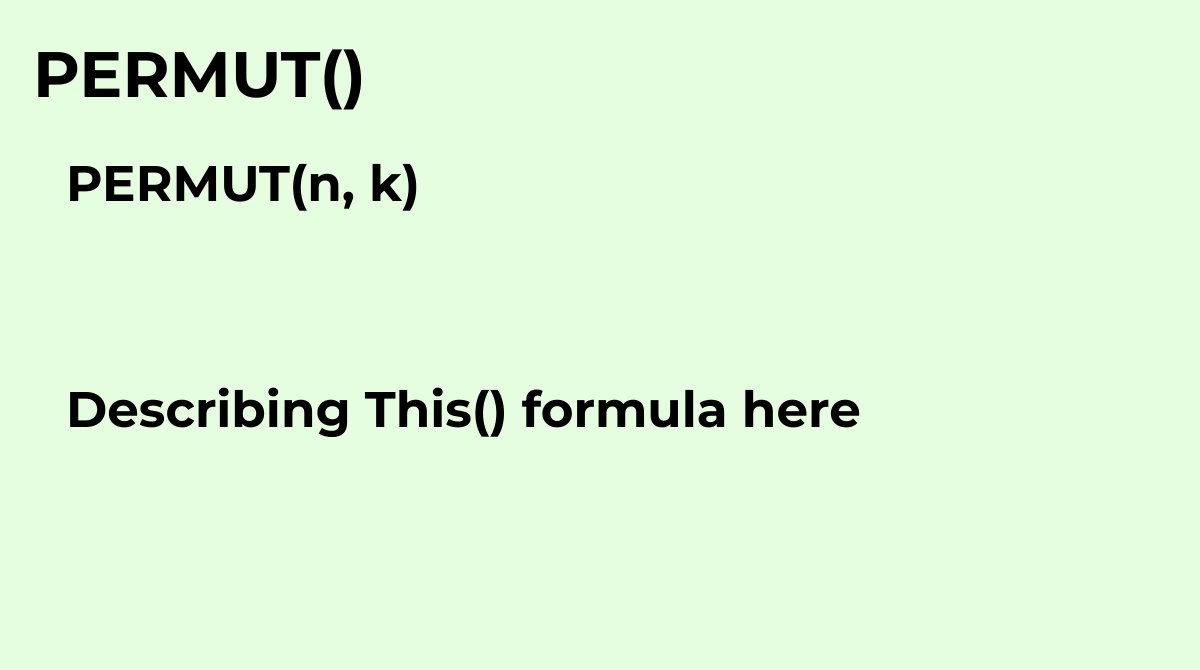Returns the number of ways to choose some number of objects from a pool of a given size of object...
`PERMUT(n, k)`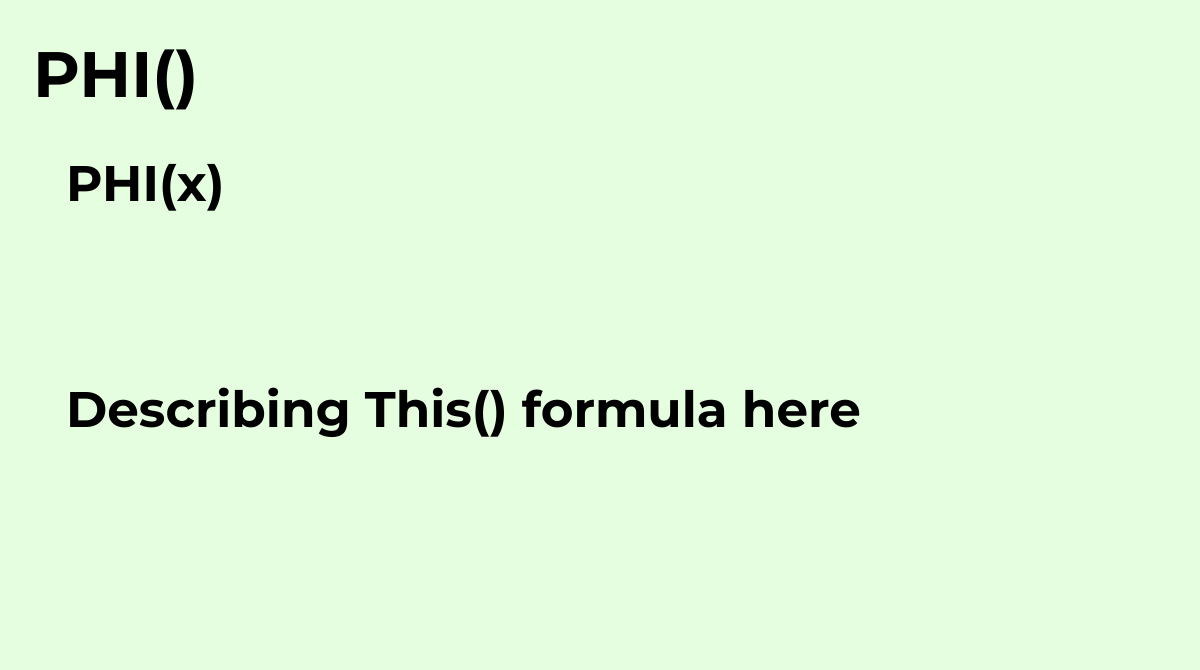The PHI function returns the value of the normal distribution with mean 0 and standard deviation 1..
`PHI(x)`

Displaying items 337-384 of 515 in total